# Frank Solutions for Class 9 Maths Chapter 14 Constructions of Triangles

Frank Solutions for Class 9 Maths Chapter 14 Constructions of Triangles consists of step by step answers to clear students’ doubts instantly. These solutions curated by teachers with years of experience are easy to understand. Students who practise these solutions regularly, gain expertise in solving tricky problems within a short duration of time. The solutions assist students to work on the important questions, which are necessary from an exam point of view. Here, students can make use of Frank Solutions for Class 9 Maths Chapter 14 Constructions of Triangles PDF free, from the links given below

Chapter 14 provides an idea of constructing triangles with step by step explanation. Students study how to construct a triangle when the lengths of all three sides are given. Expert faculty prepare these solutions to help students in the exams.

## Frank Solutions for Class 9 Maths Chapter 14 Construction of Triangles Download PDF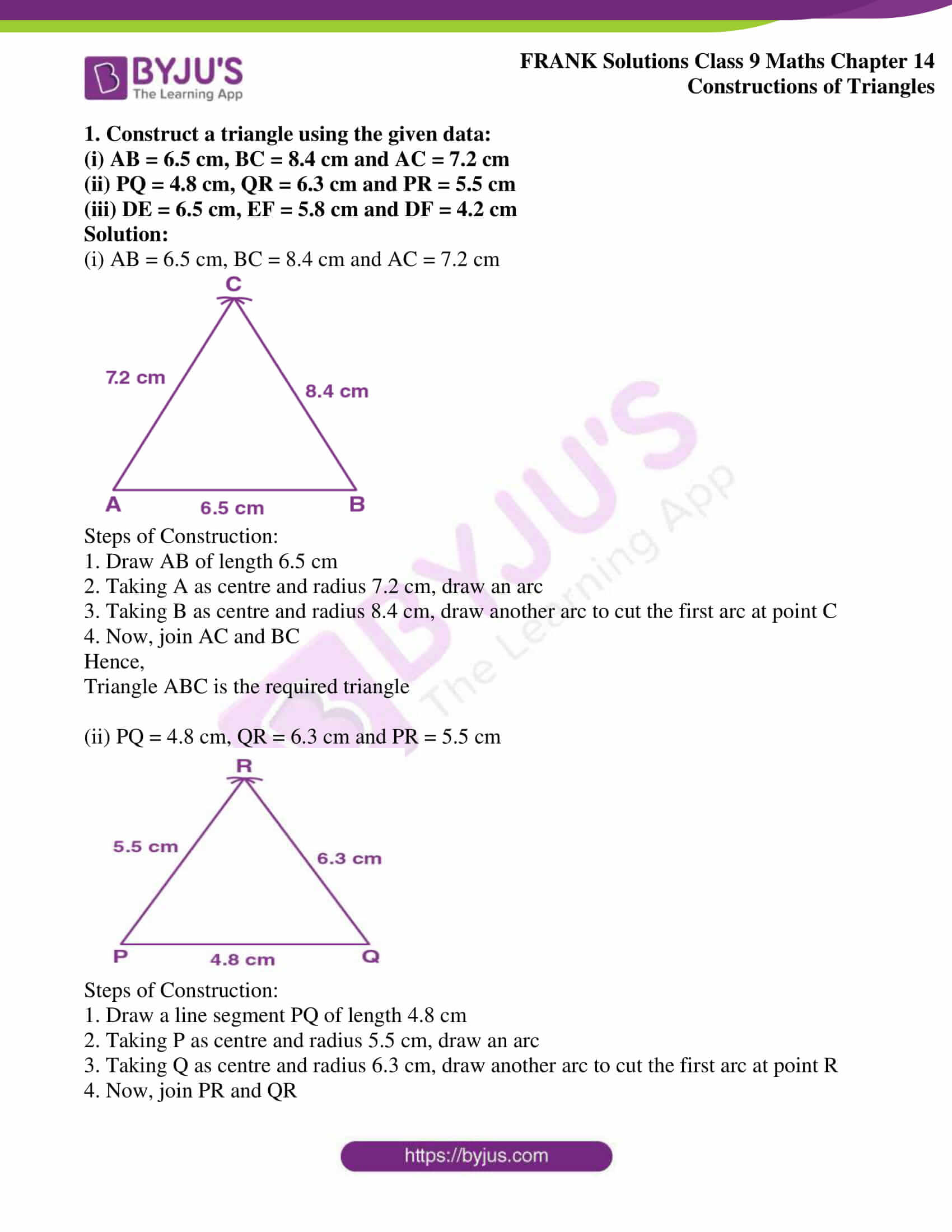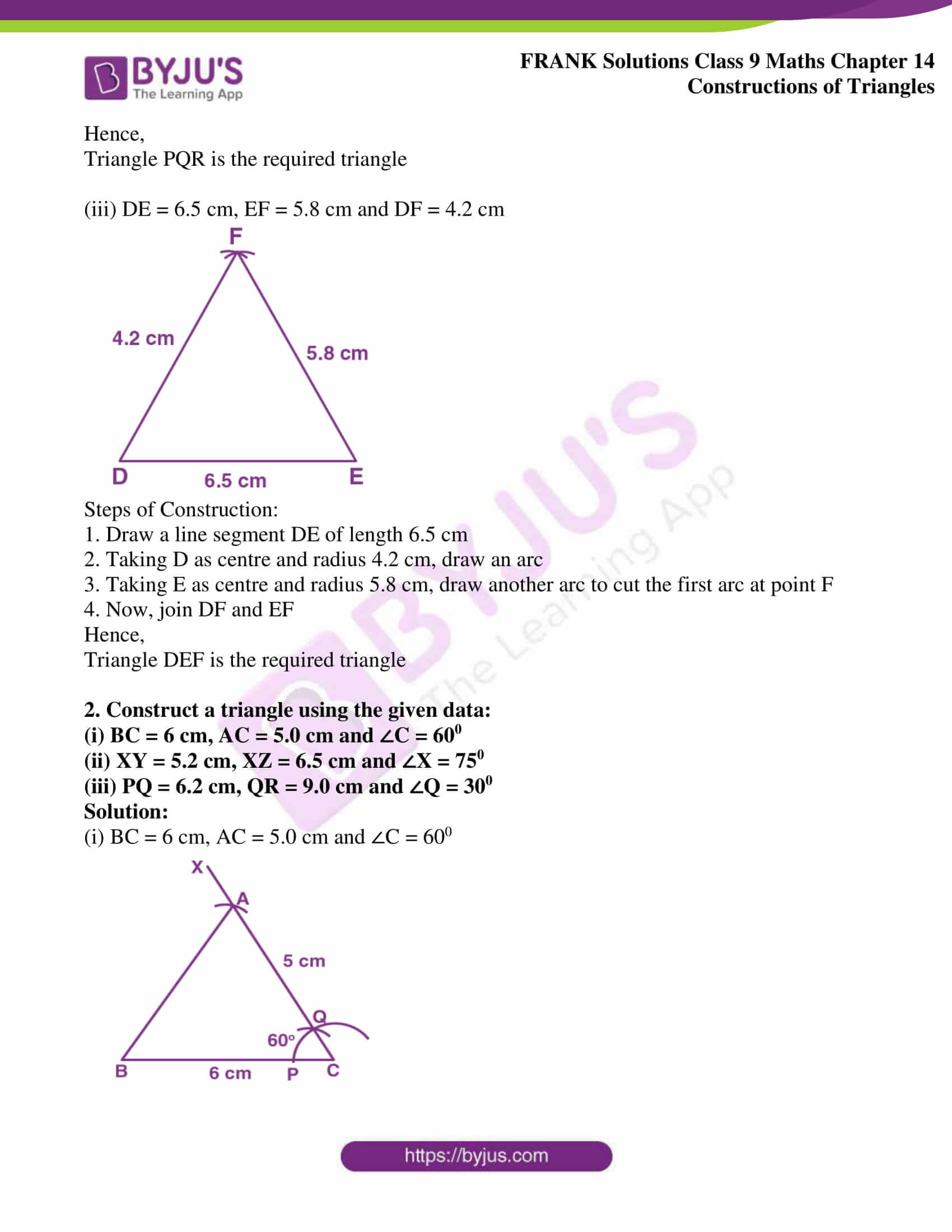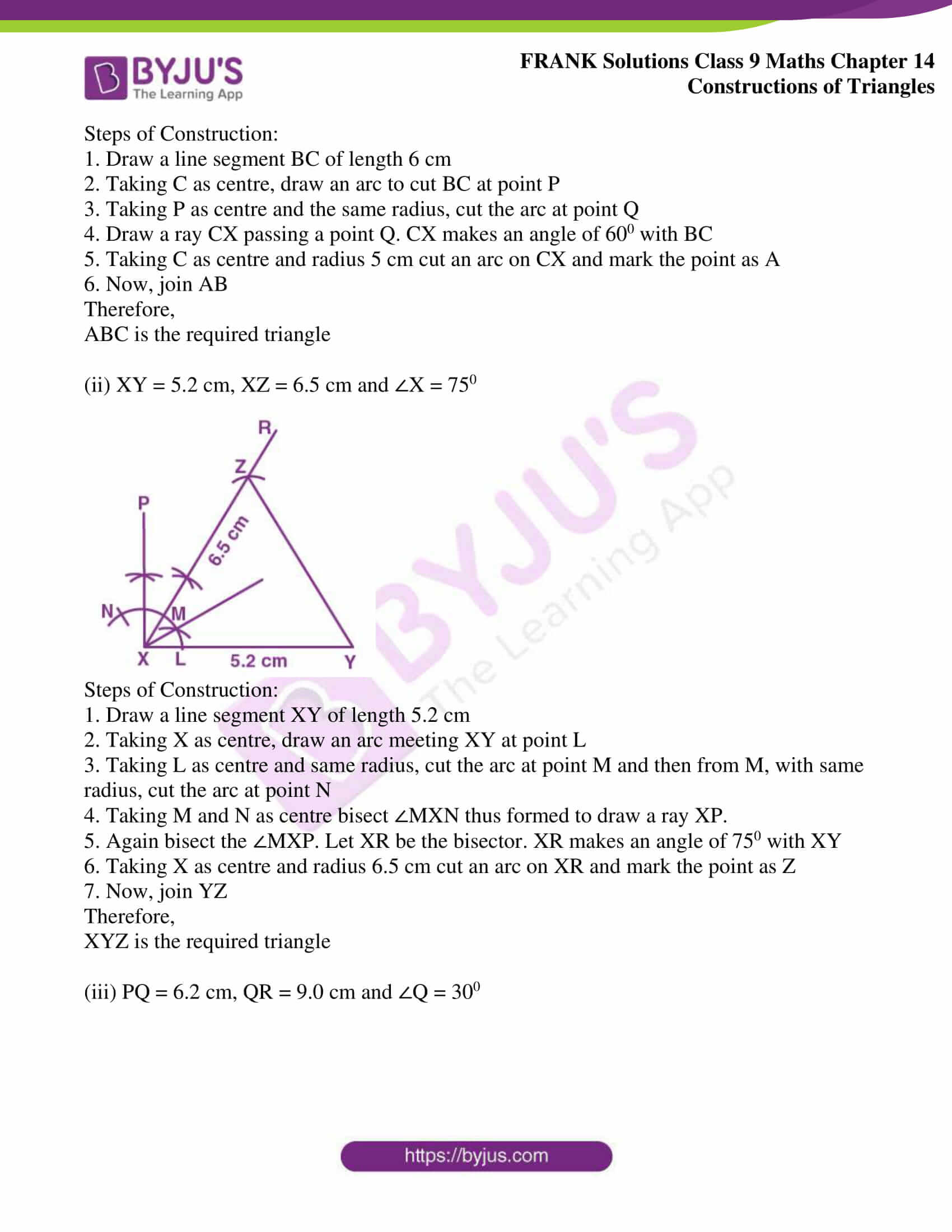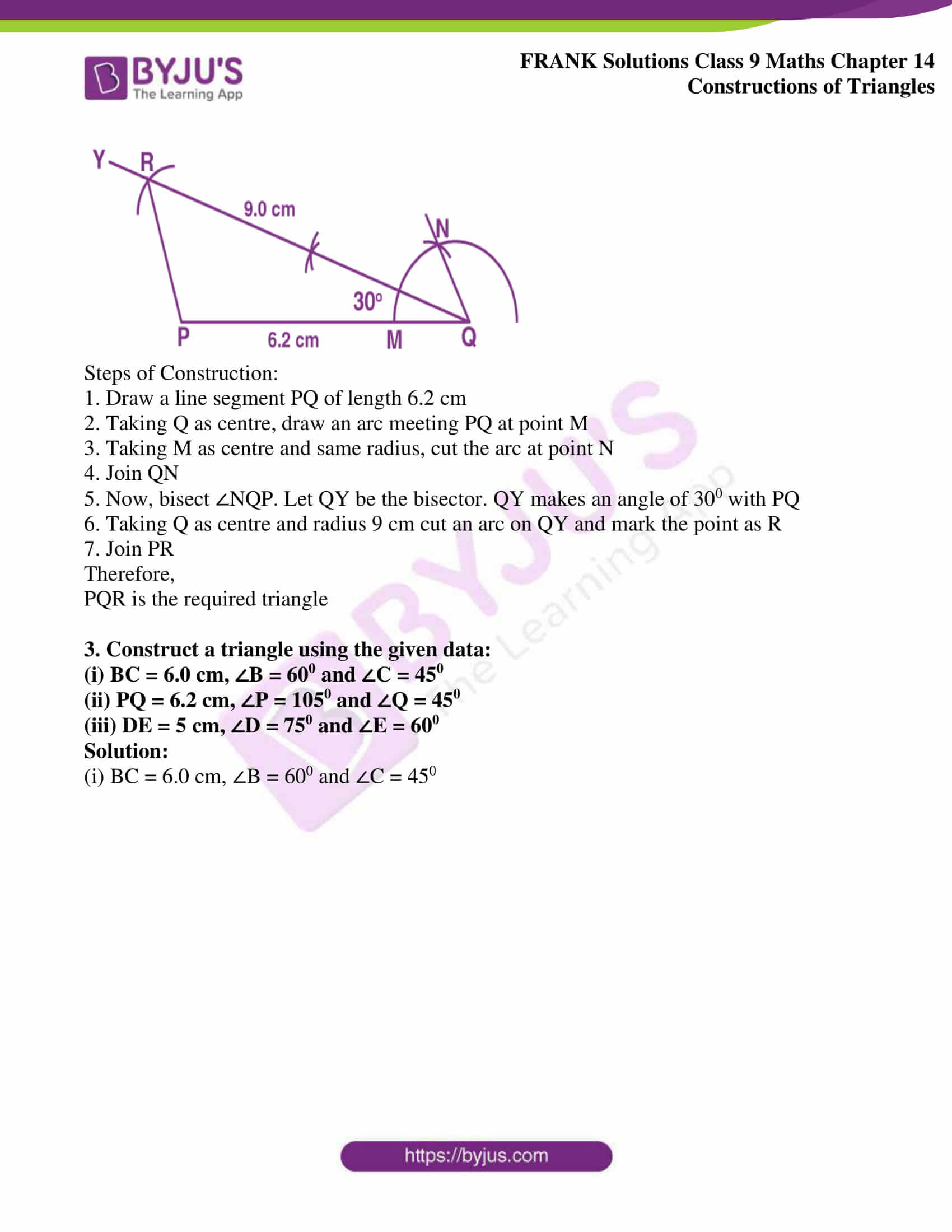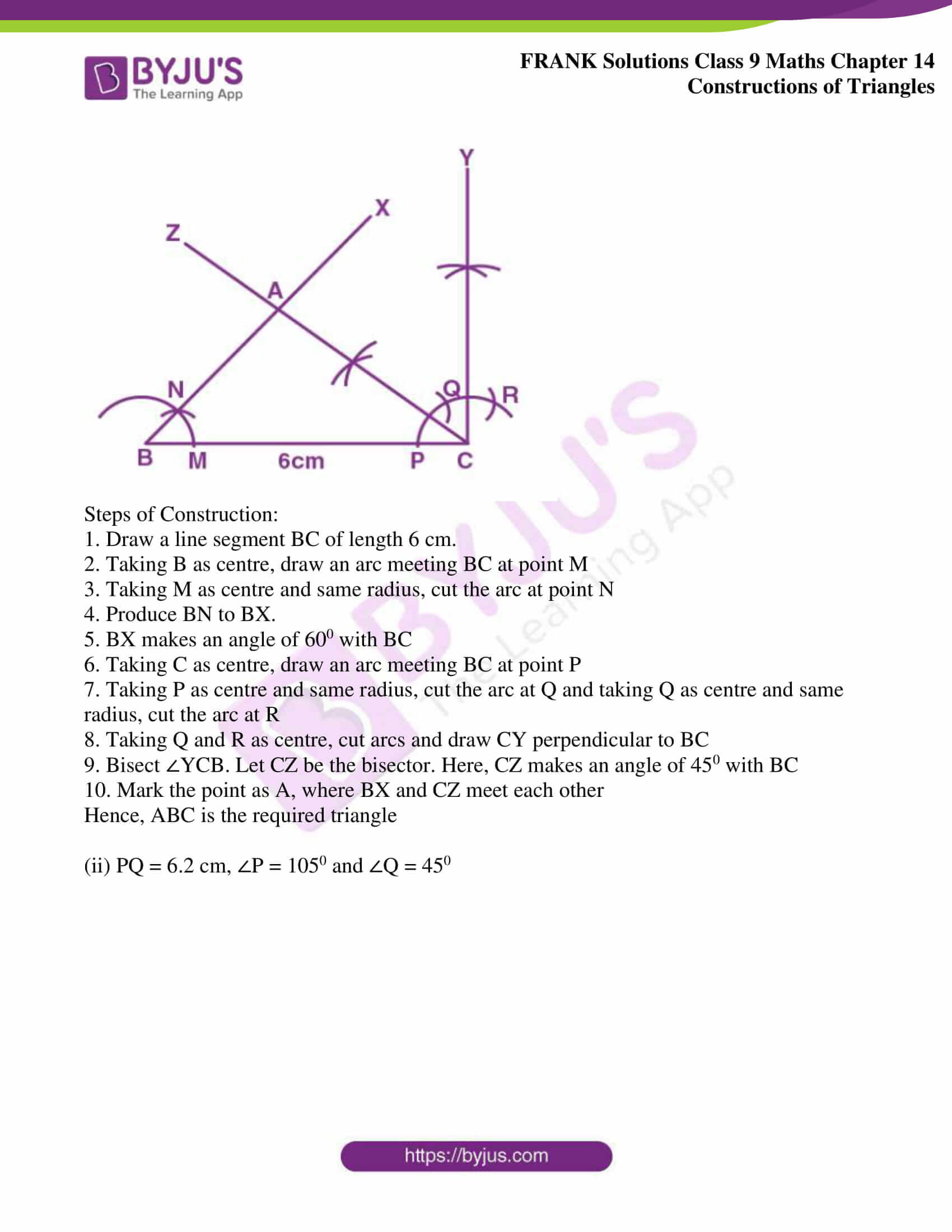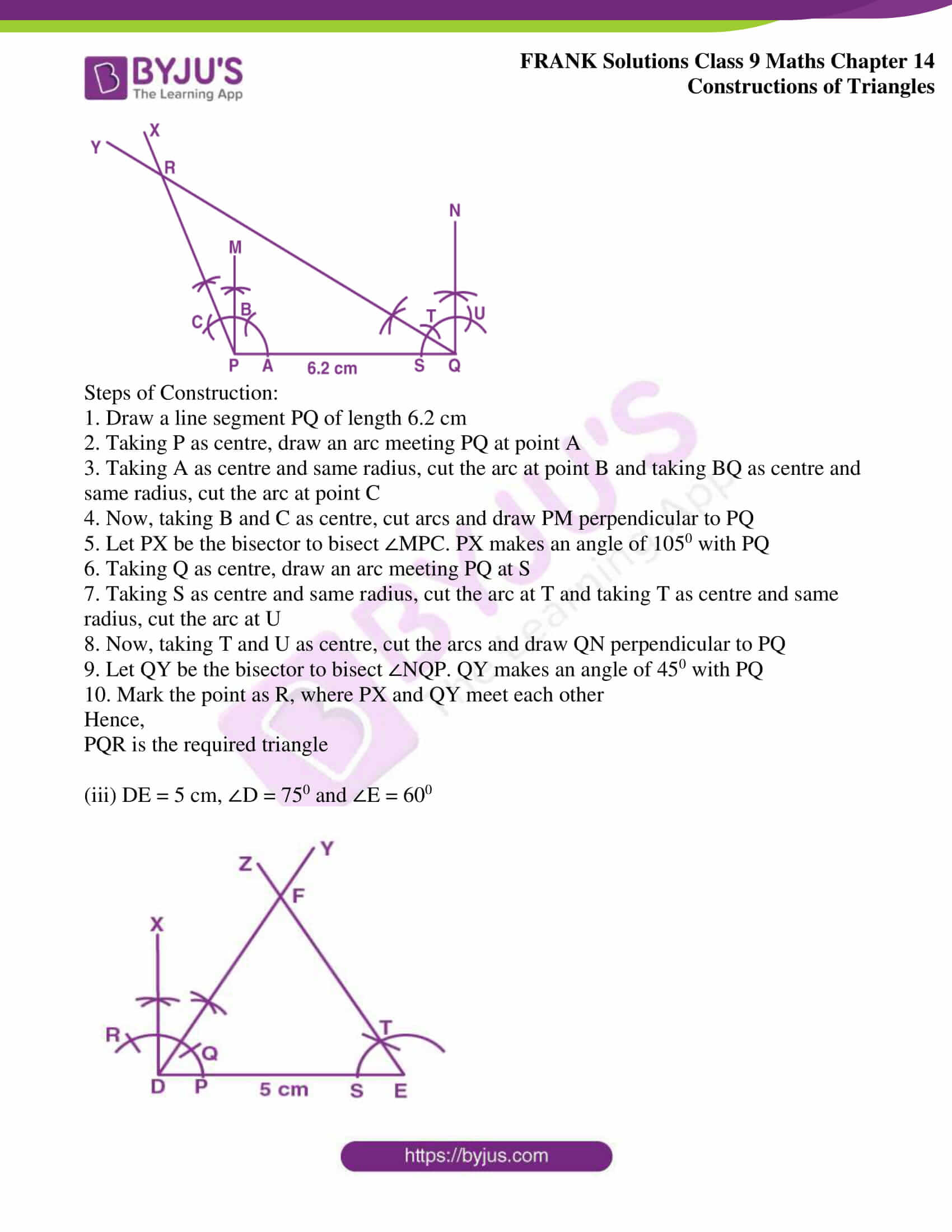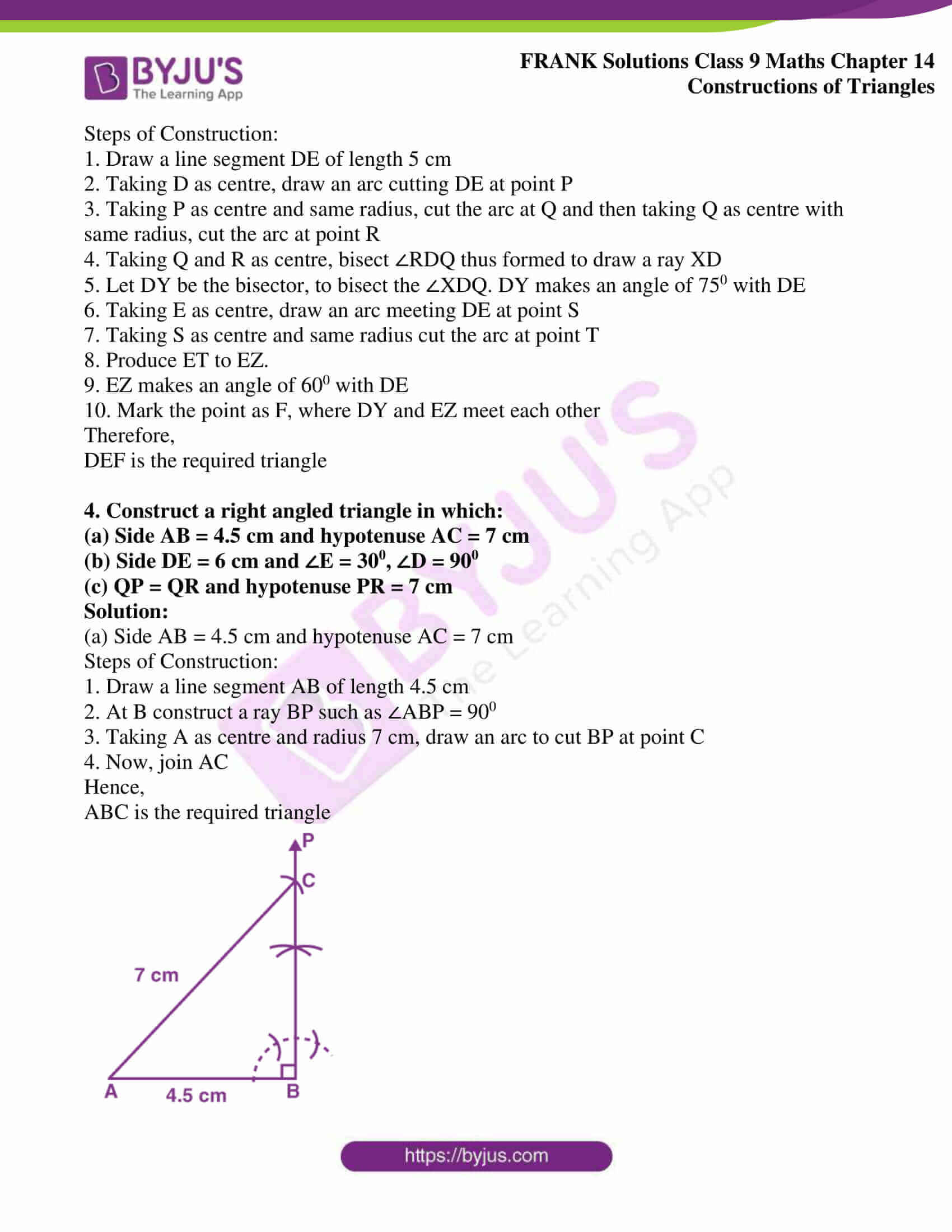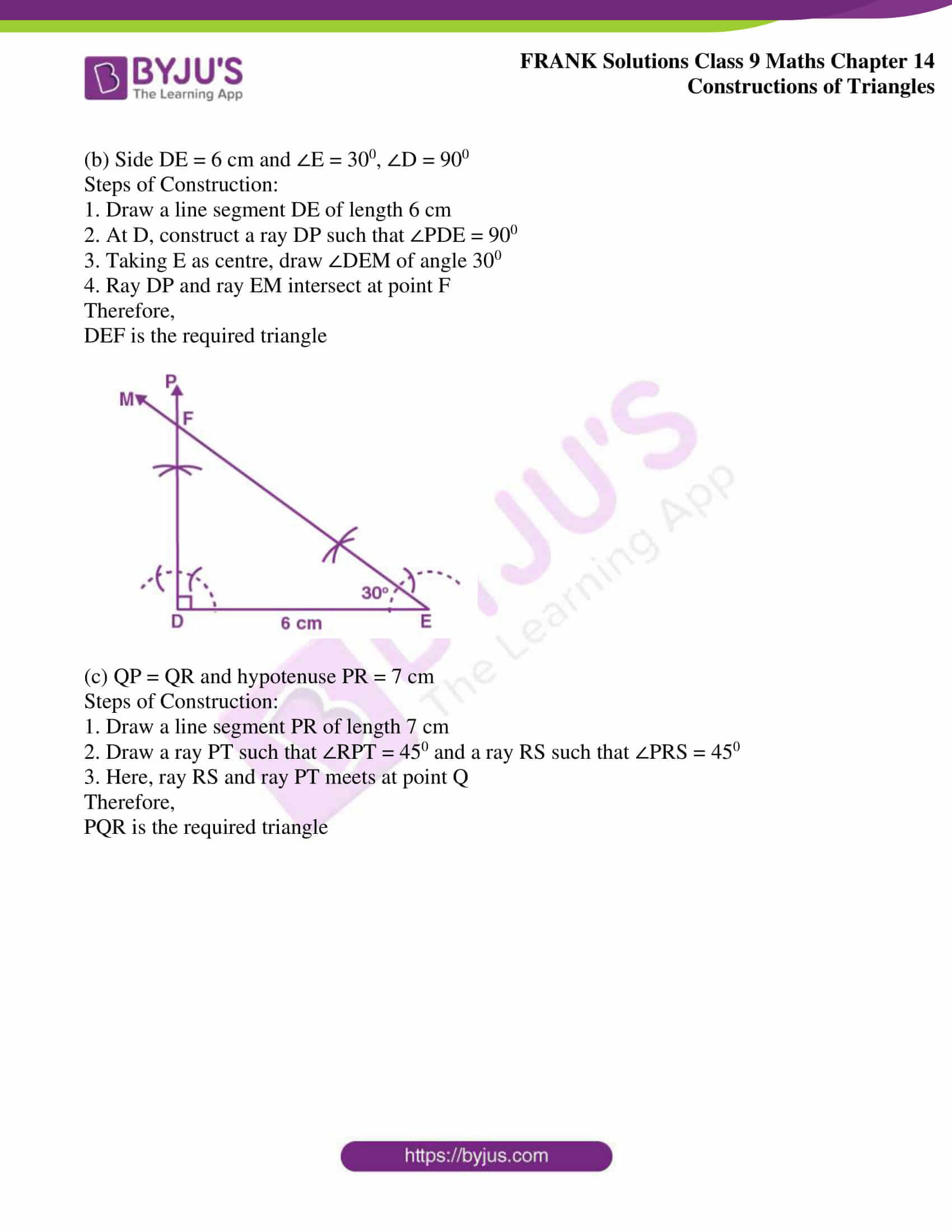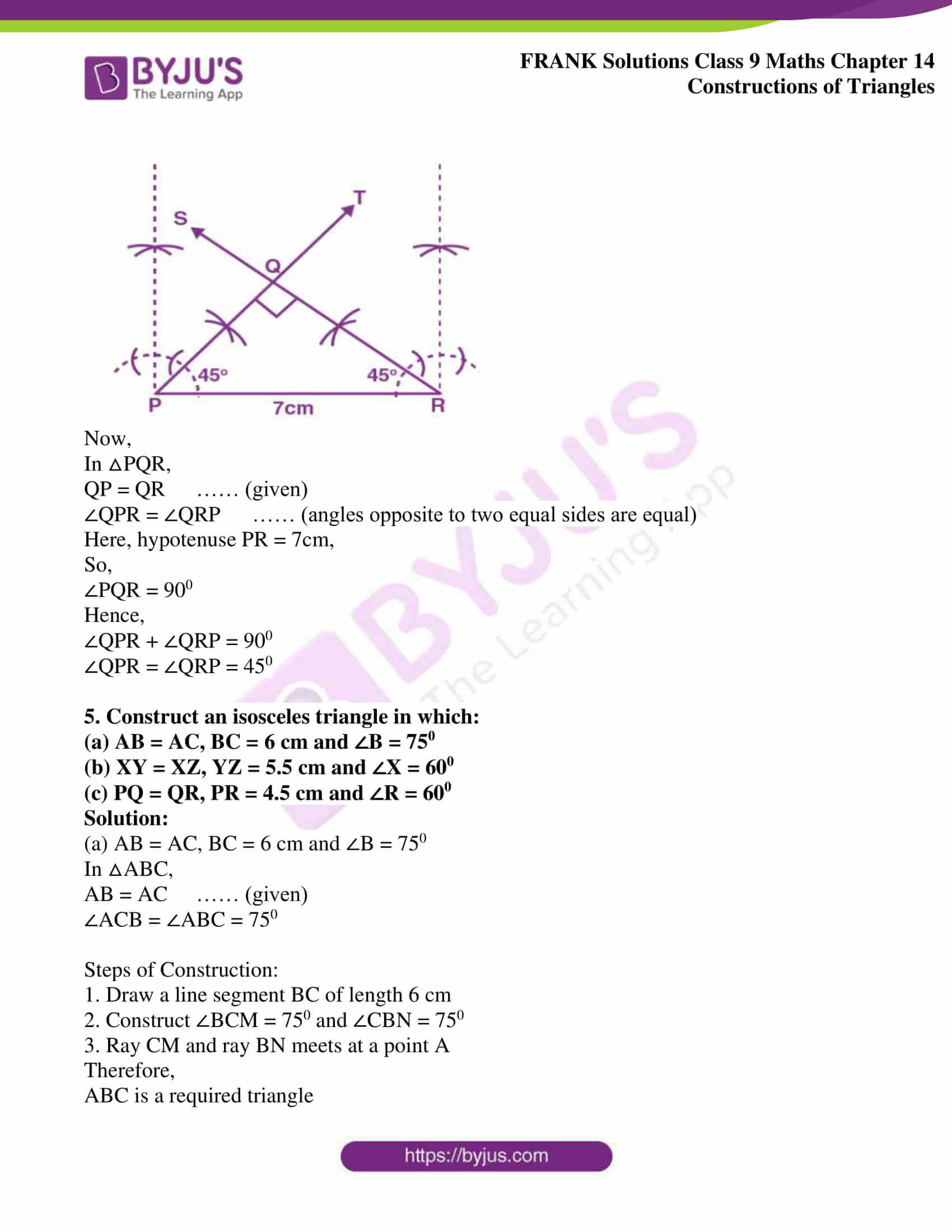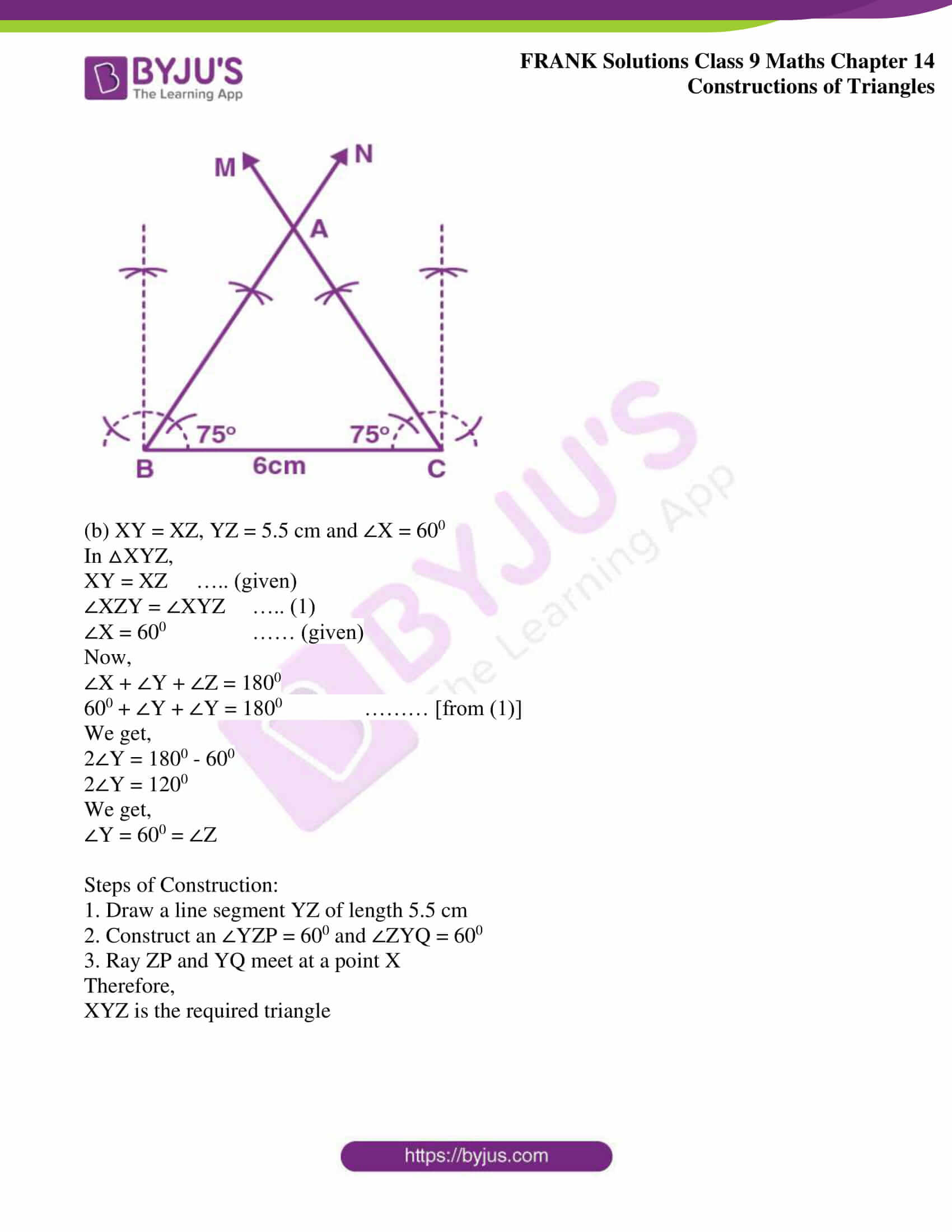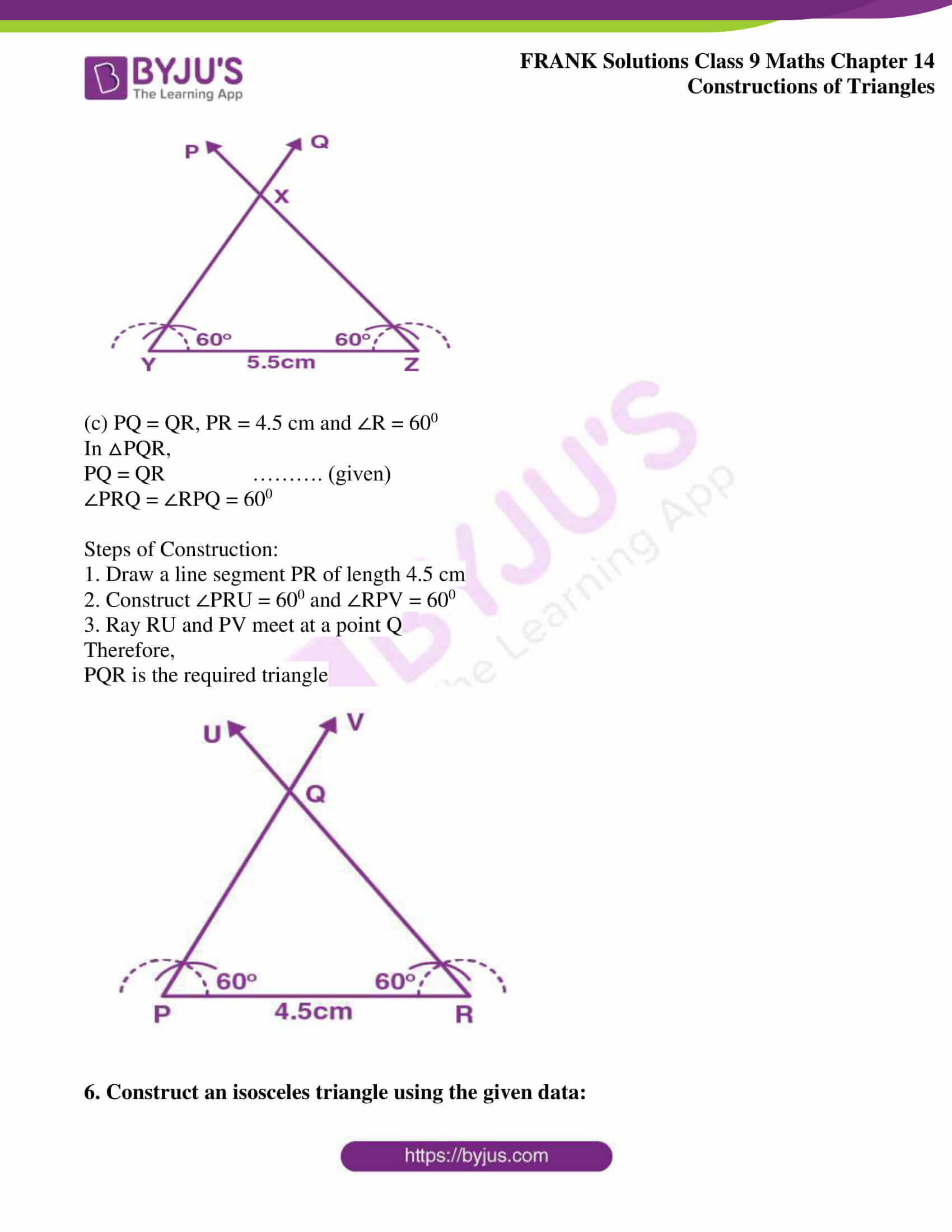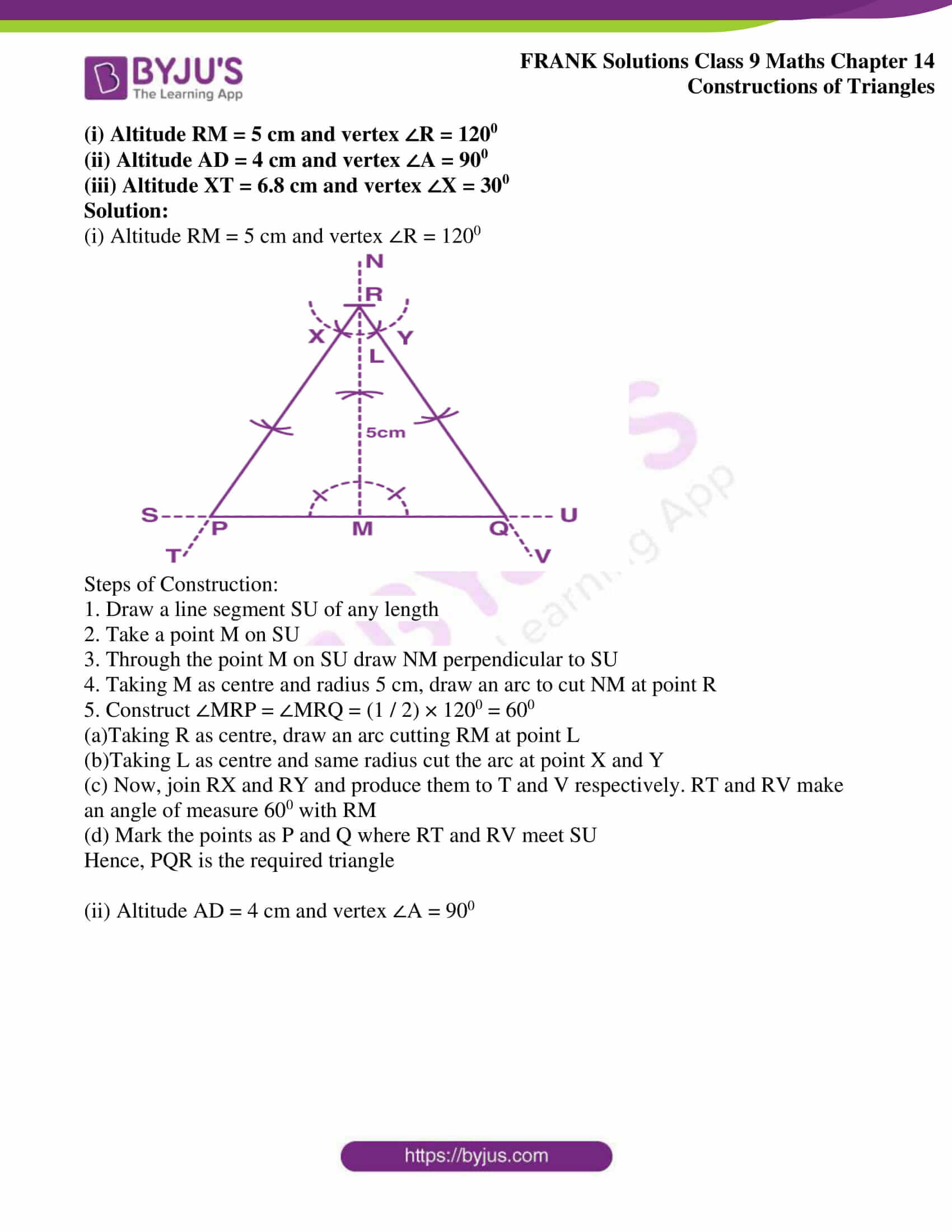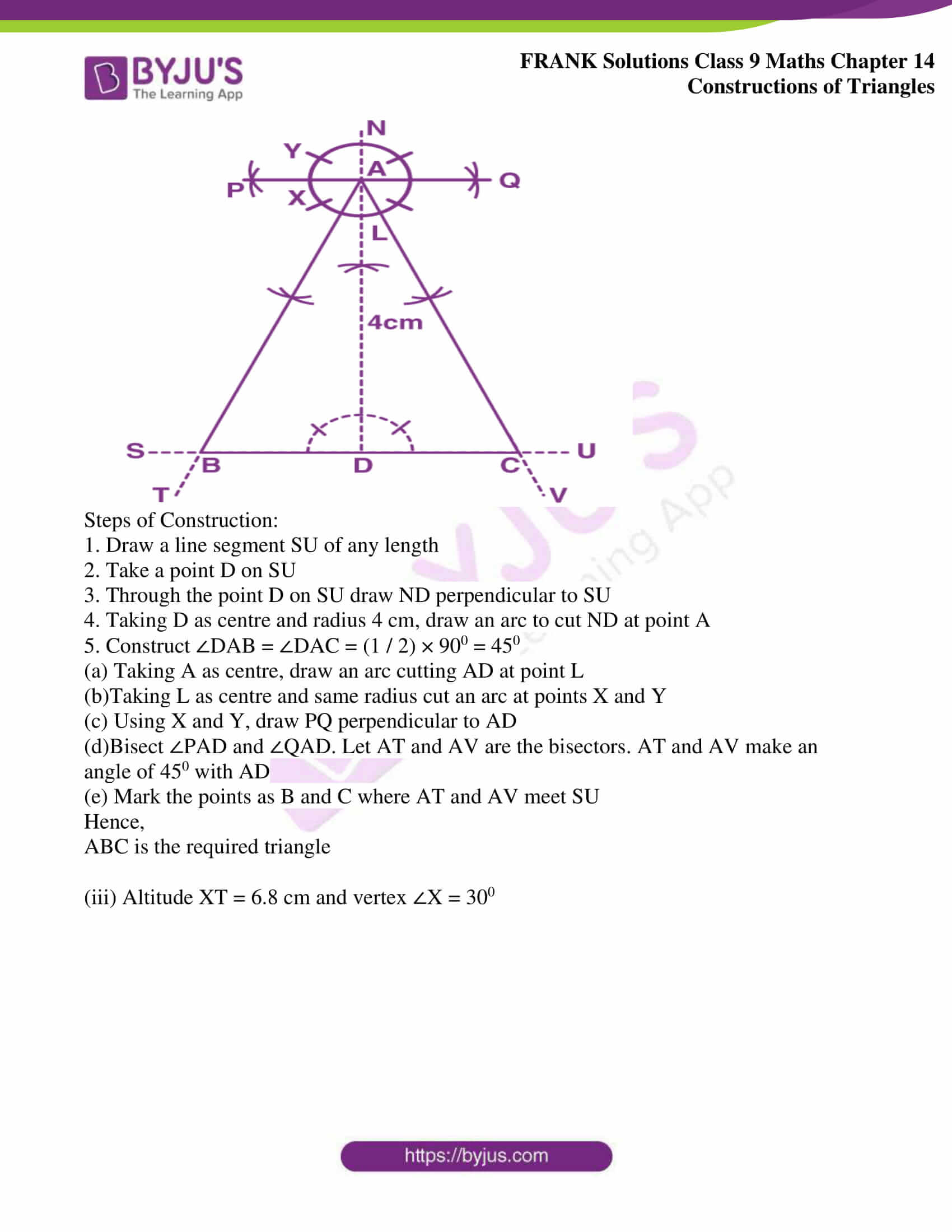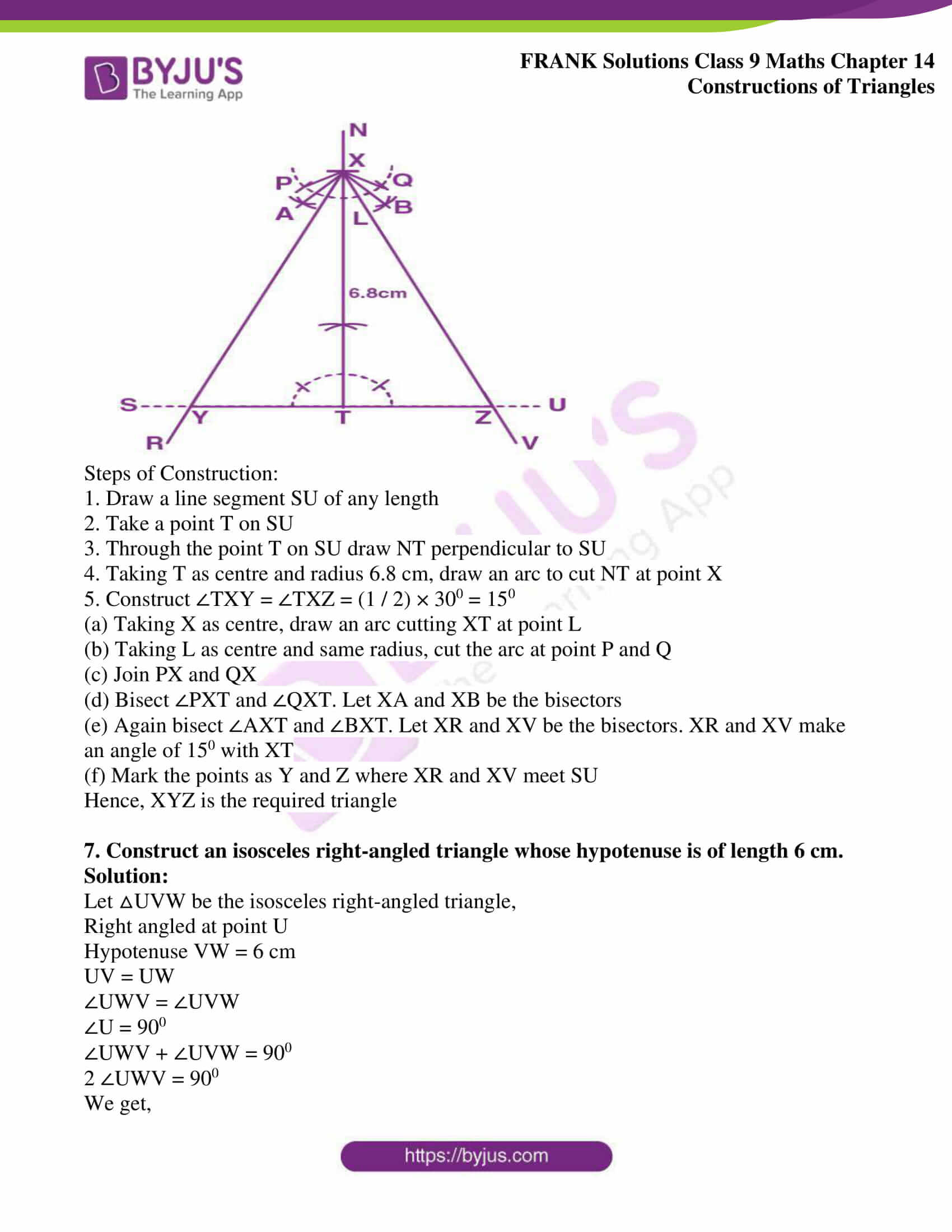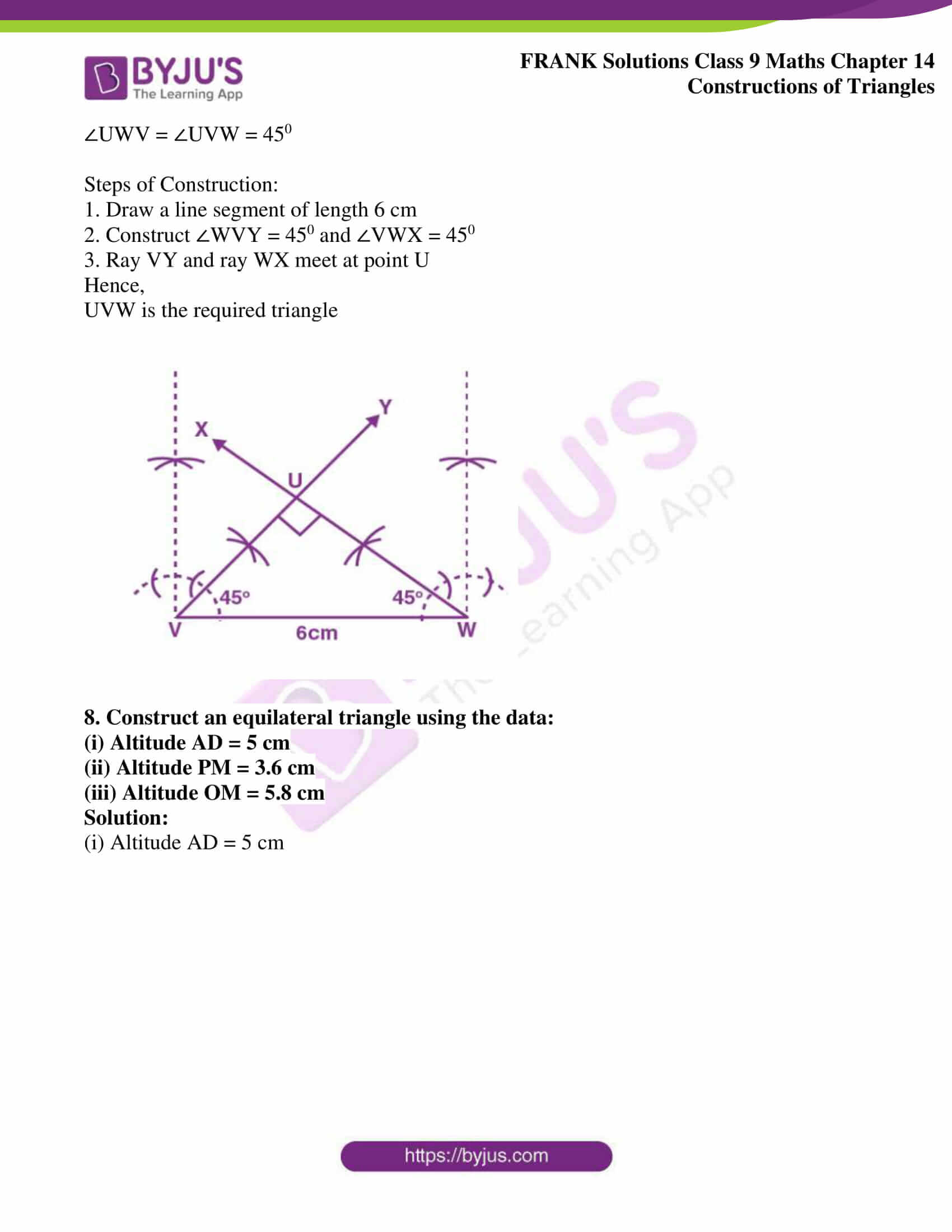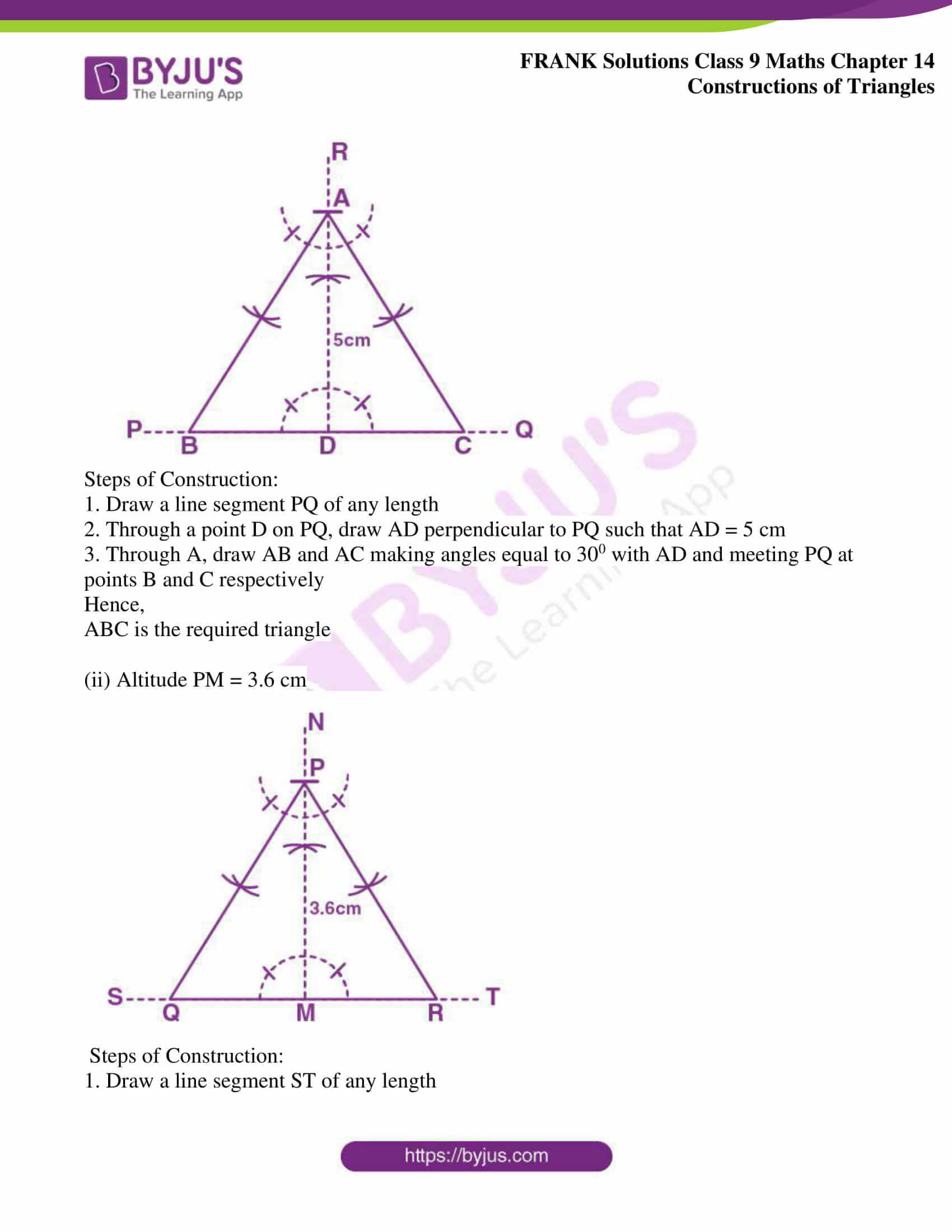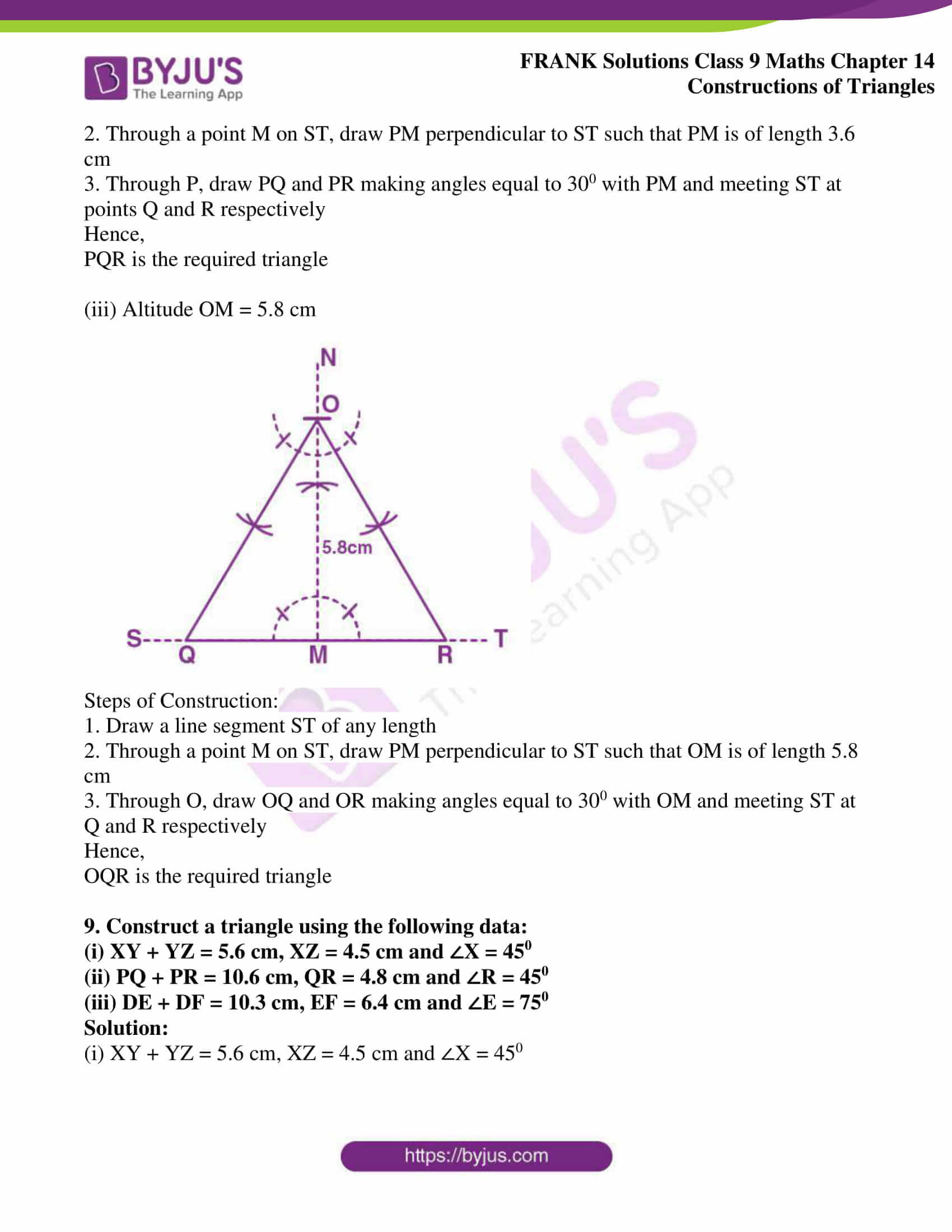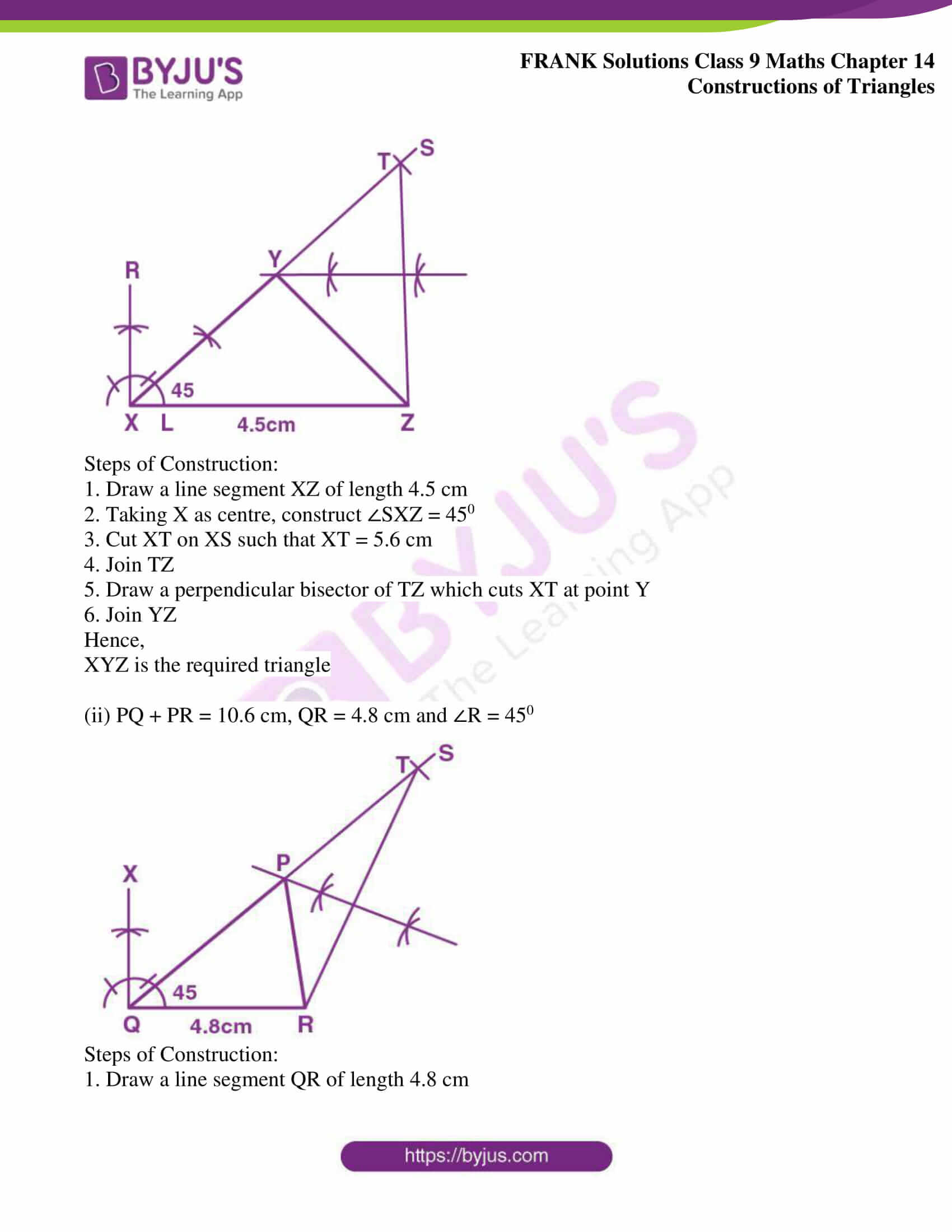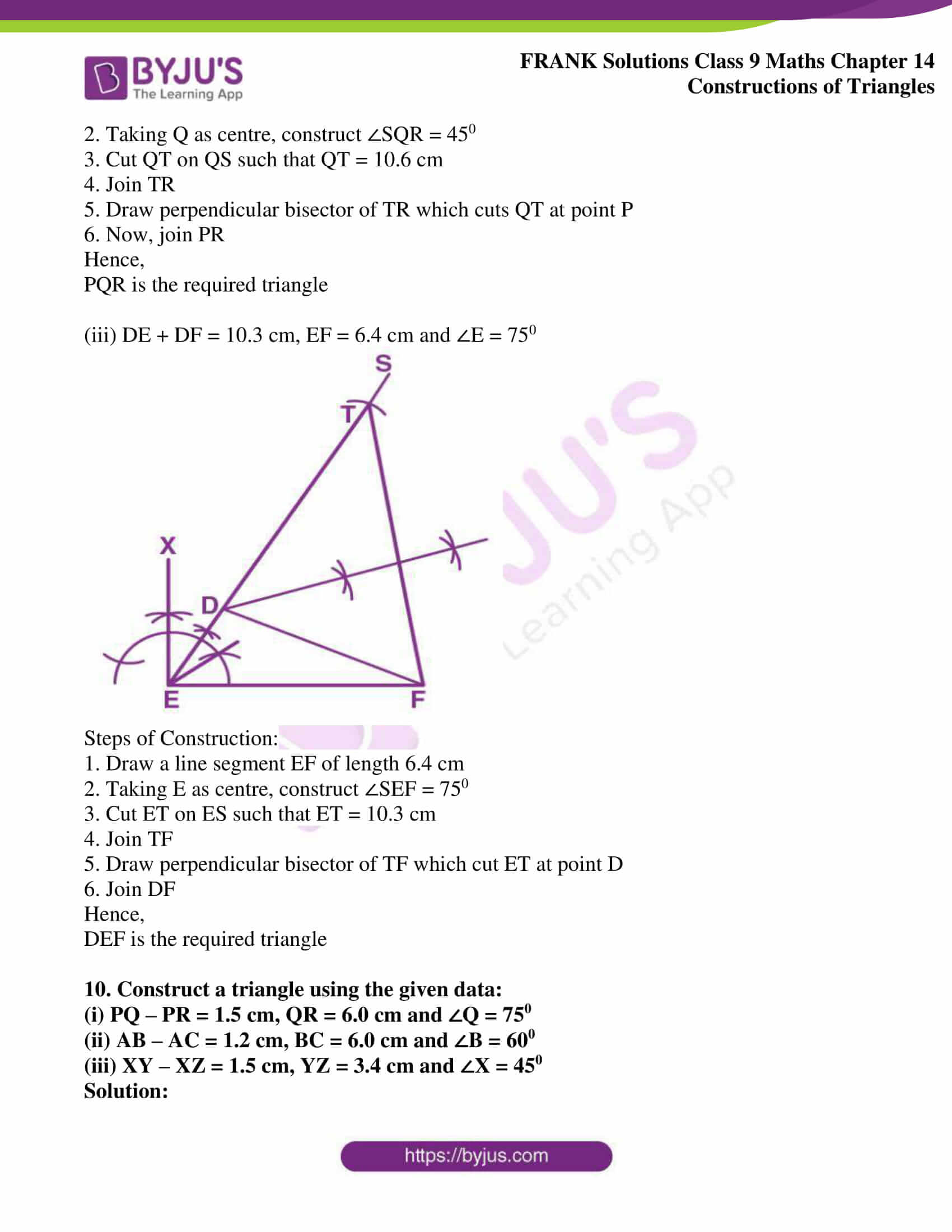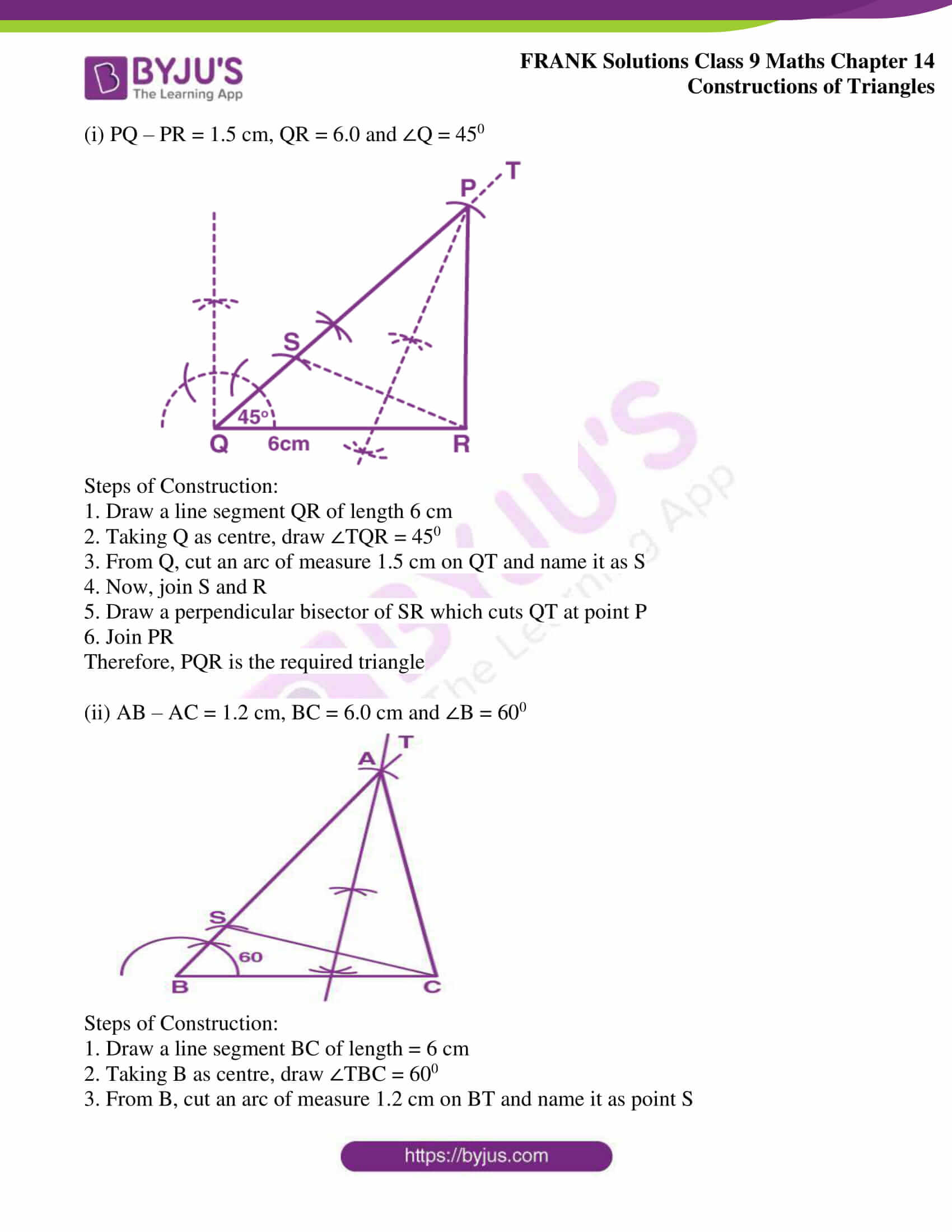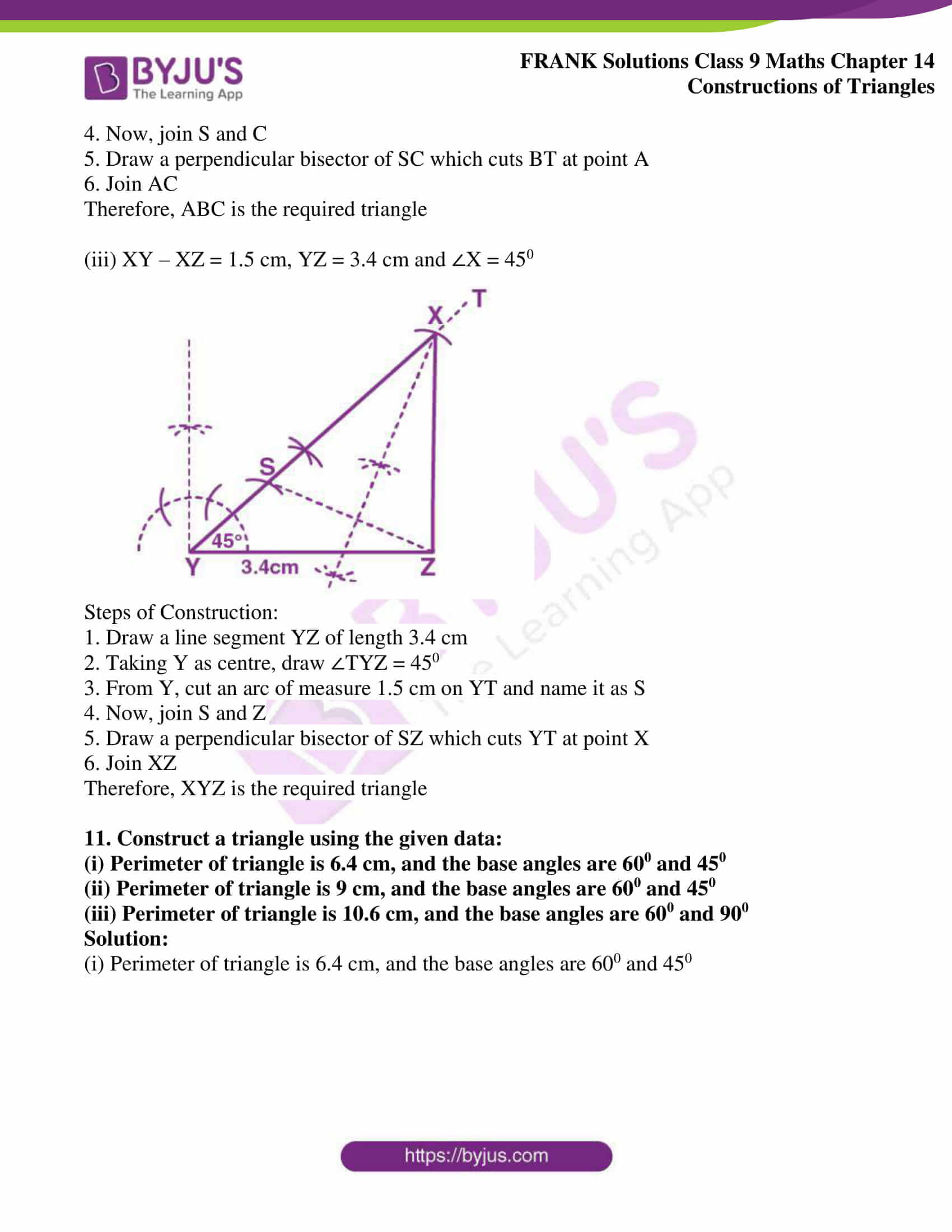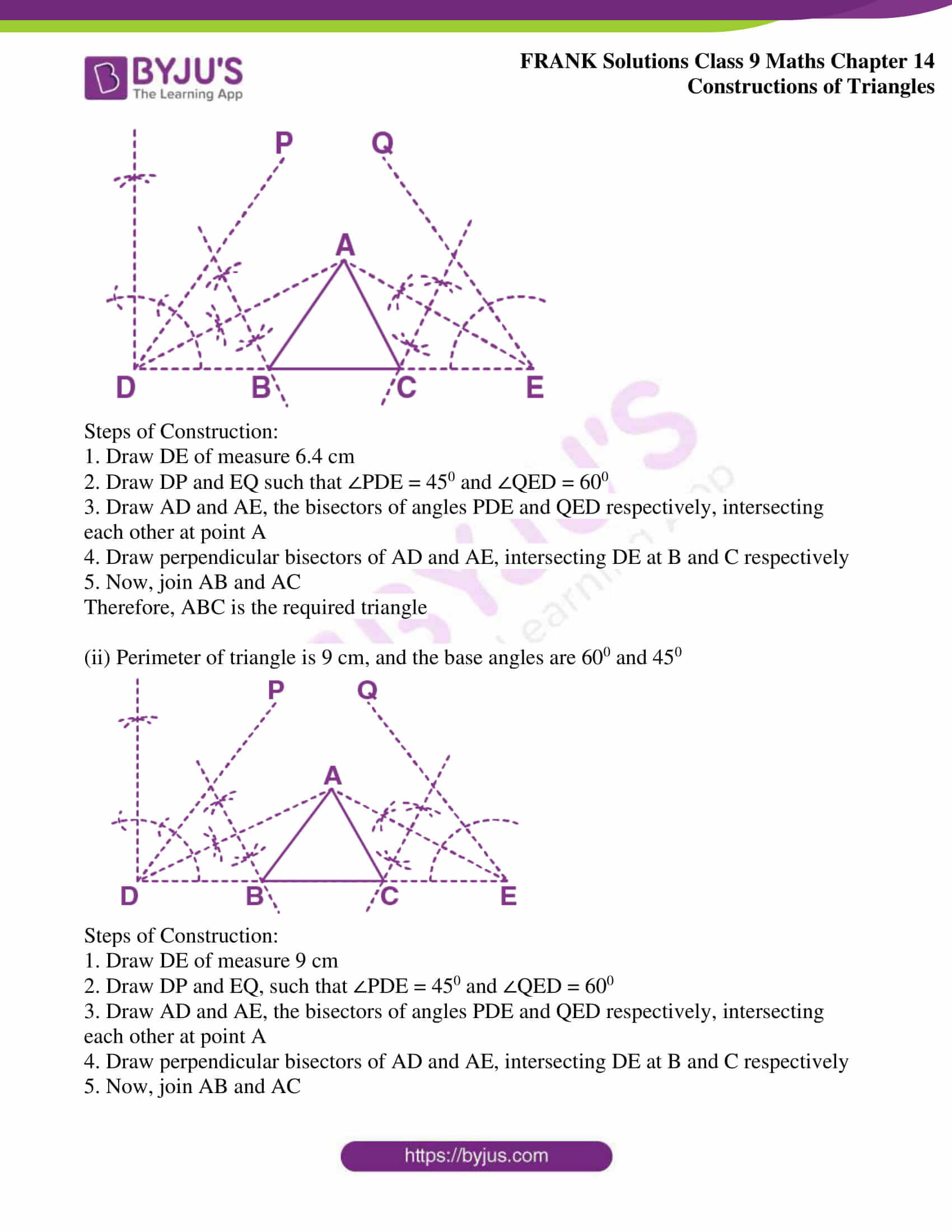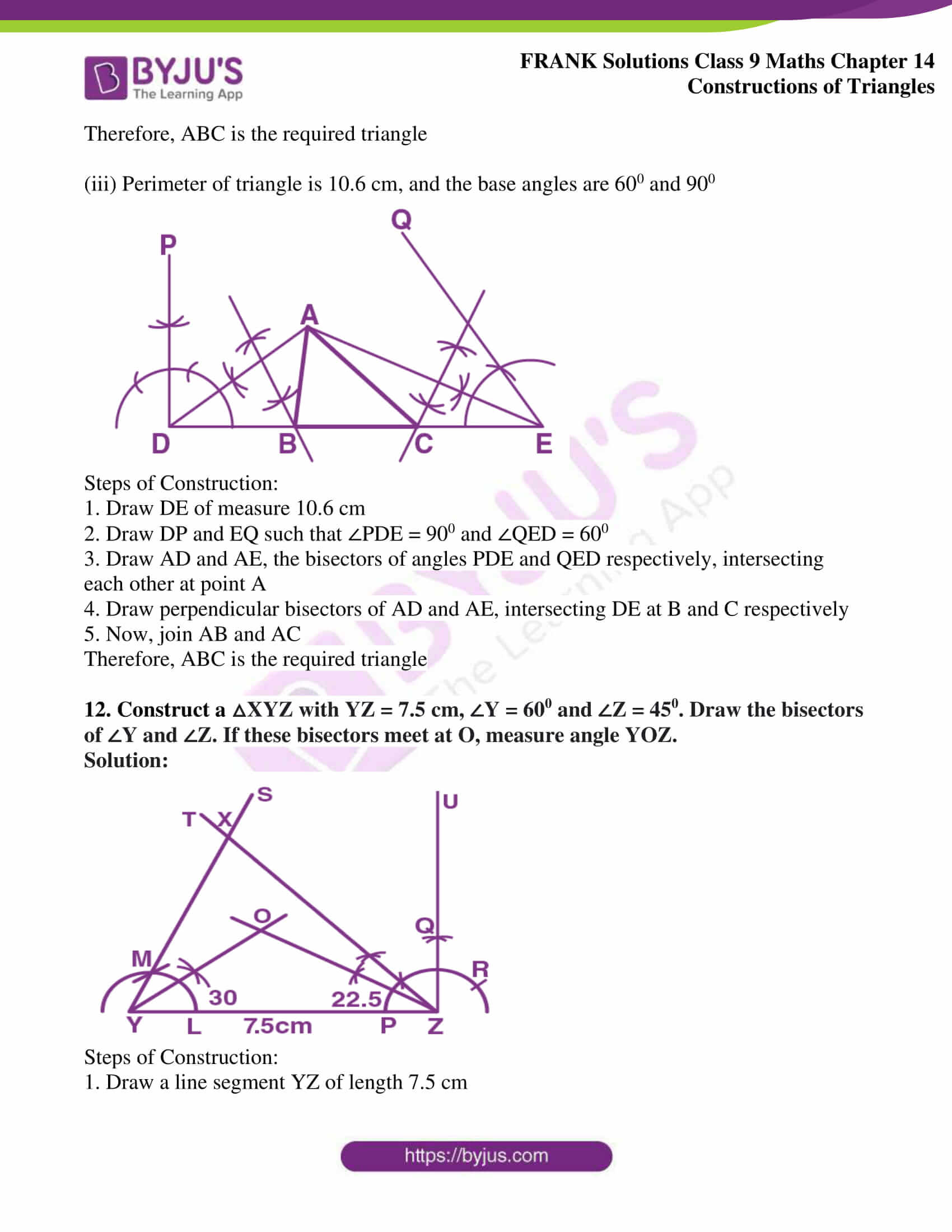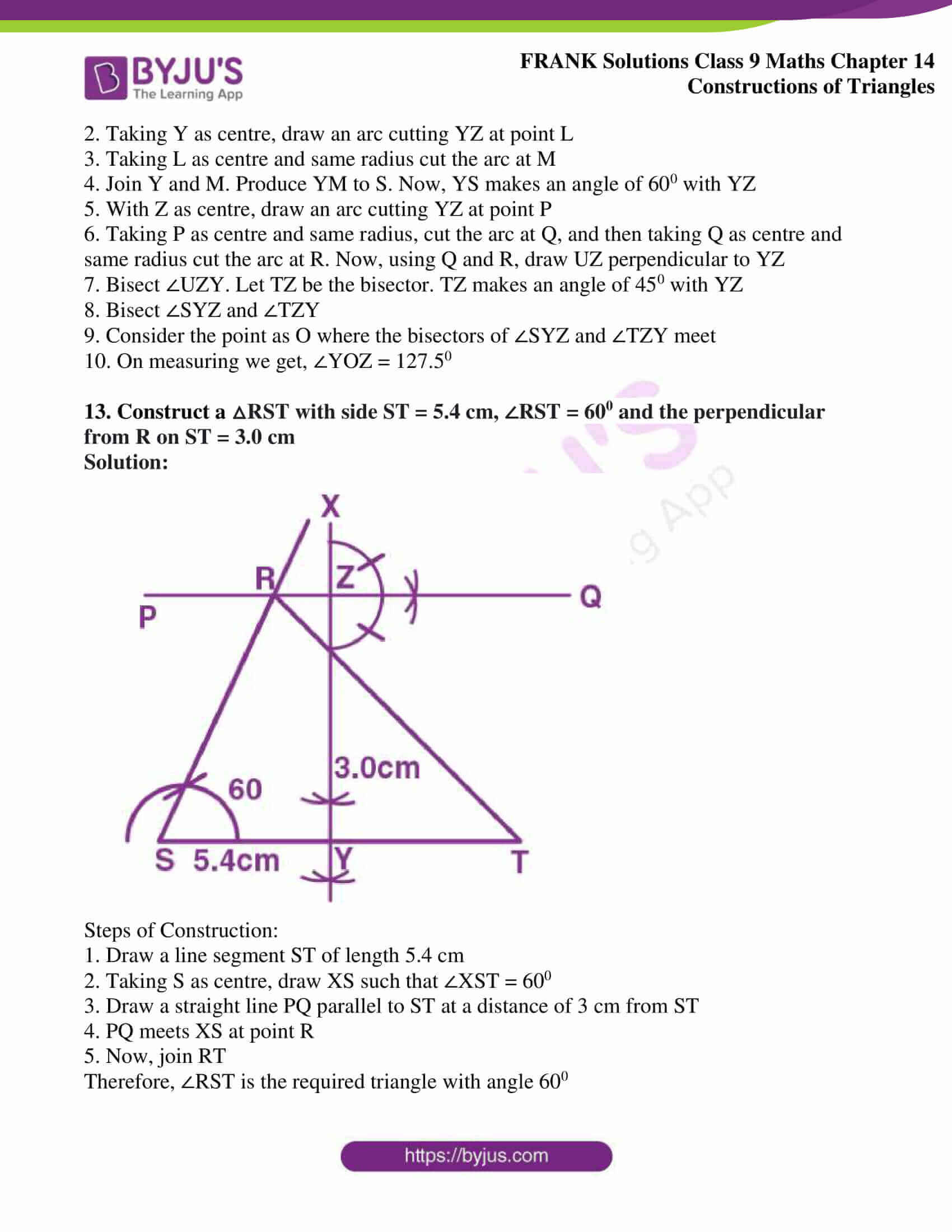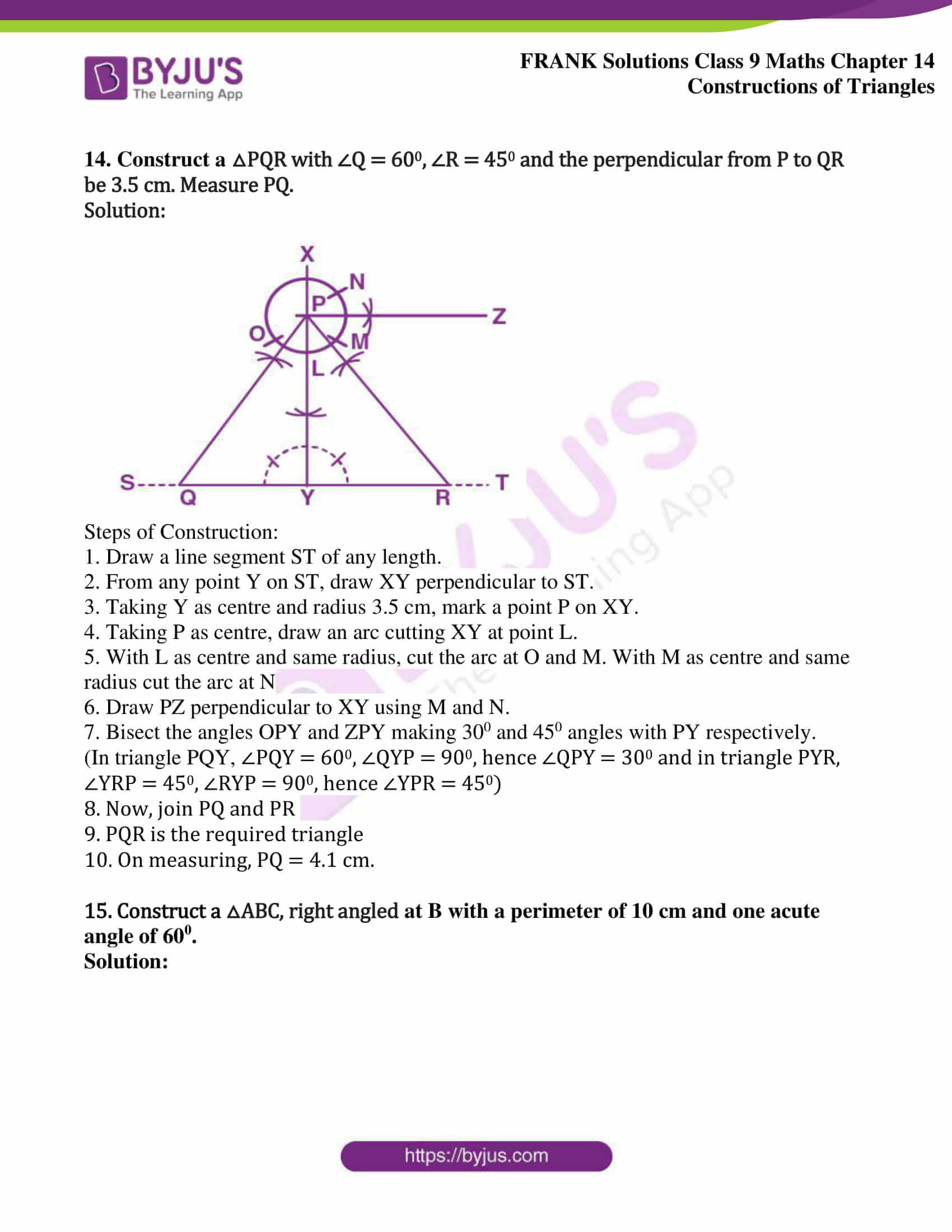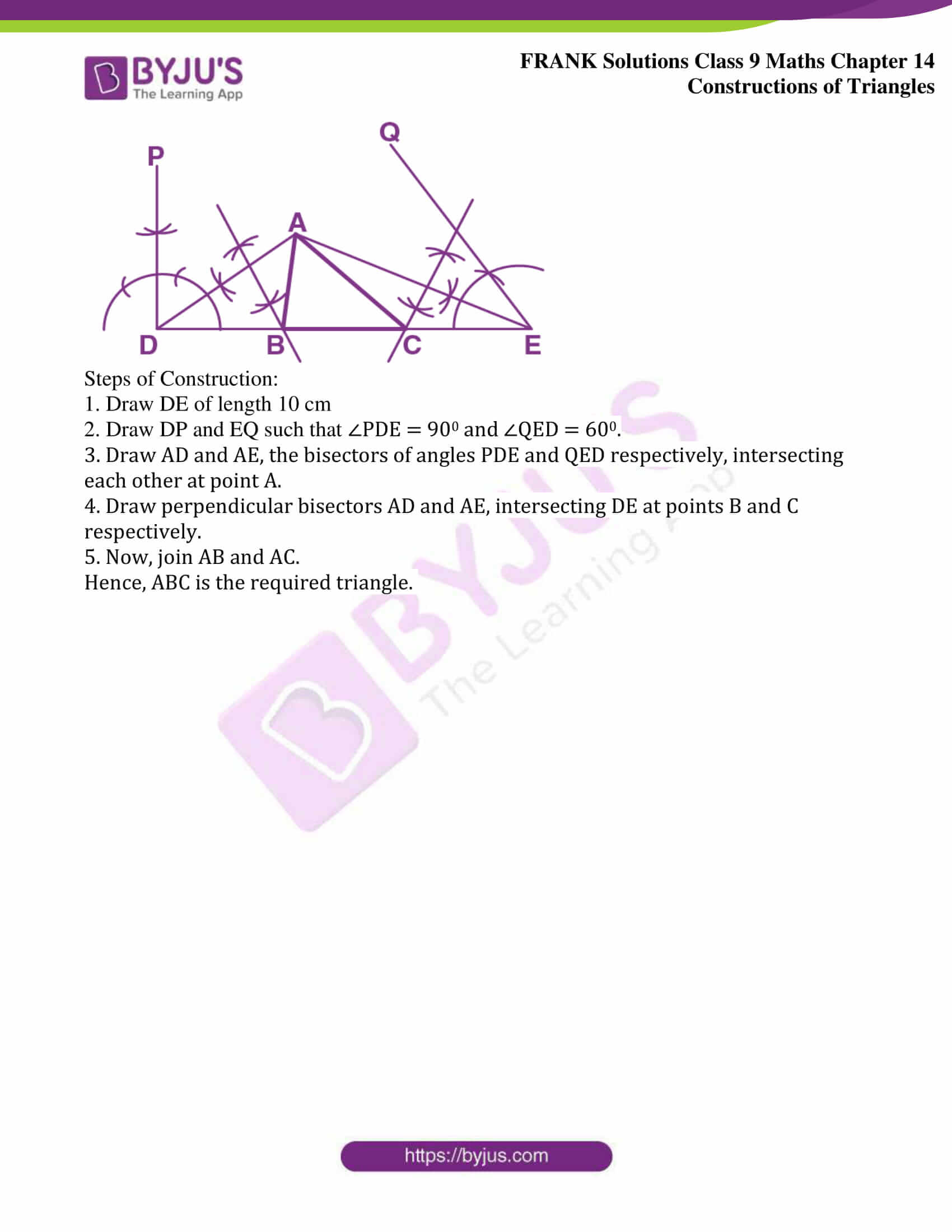## Access Frank Solutions for Class 9 Maths Chapter 14 Construction of Triangles

1. Construct a triangle using the given data:

(i) AB = 6.5 cm, BC = 8.4 cm and AC = 7.2 cm

(ii) PQ = 4.8 cm, QR = 6.3 cm and PR = 5.5 cm

(iii) DE = 6.5 cm, EF = 5.8 cm and DF = 4.2 cm

Solution:

(i) AB = 6.5 cm, BC = 8.4 cm and AC = 7.2 cm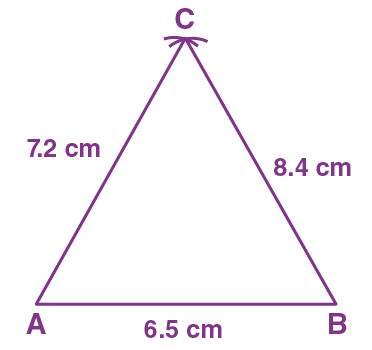Steps of Construction:

1. Draw AB of length 6.5 cm

2. Taking A as centre and radius 7.2 cm, draw an arc

3. Taking B as centre and radius 8.4 cm, draw another arc to cut the first arc at point C

4. Now, join AC and BC

Hence,

Triangle ABC is the required triangle

(ii) PQ = 4.8 cm, QR = 6.3 cm and PR = 5.5 cm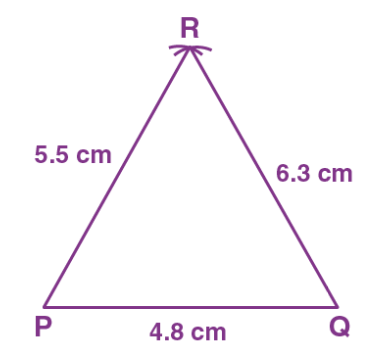Steps of Construction:

1. Draw a line segment PQ of length 4.8 cm

2. Taking P as centre and radius 5.5 cm, draw an arc

3. Taking Q as centre and radius 6.3 cm, draw another arc to cut the first arc at point R

4. Now, join PR and QR

Hence,

Triangle PQR is the required triangle

(iii) DE = 6.5 cm, EF = 5.8 cm and DF = 4.2 cm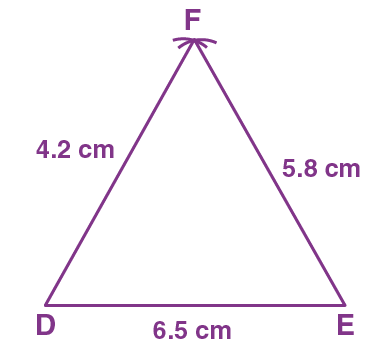Steps of Construction:

1. Draw a line segment DE of length 6.5 cm

2. Taking D as centre and radius 4.2 cm, draw an arc

3. Taking E as centre and radius 5.8 cm, draw another arc to cut the first arc at point F

4. Now, join DF and EF

Hence,

Triangle DEF is the required triangle

2. Construct a triangle using the given data:

(i) BC = 6 cm, AC = 5.0 cm and ∠C = 600

(ii) XY = 5.2 cm, XZ = 6.5 cm and ∠X = 750

(iii) PQ = 6.2 cm, QR = 9.0 cm and ∠Q = 300

Solution:

(i) BC = 6 cm, AC = 5.0 cm and ∠C = 600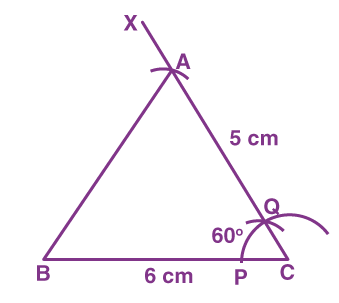Steps of Construction:

1. Draw a line segment BC of length 6 cm

2. Taking C as centre, draw an arc to cut BC at point P

3. Taking P as centre and the same radius, cut the arc at point Q

4. Draw a ray CX passing a point Q. CX makes an angle of 600 with BC

5. Taking C as centre and radius 5 cm cut an arc on CX and mark the point as A

6. Now, join AB

Therefore,

ABC is the required triangle

(ii) XY = 5.2 cm, XZ = 6.5 cm and ∠X = 750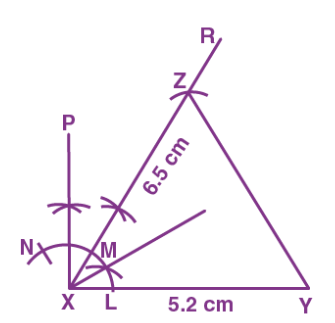Steps of Construction:

1. Draw a line segment XY of length 5.2 cm

2. Taking X as centre, draw an arc meeting XY at point L

3. Taking L as centre and same radius, cut the arc at point M and then from M, with same radius, cut the arc at point N

4. Taking M and N as centre bisect ∠MXN thus formed to draw a ray XP.

5. Again bisect the ∠MXP. Let XR be the bisector. XR makes an angle of 750 with XY

6. Taking X as centre and radius 6.5 cm cut an arc on XR and mark the point as Z

7. Now, join YZ

Therefore,

XYZ is the required triangle

(iii) PQ = 6.2 cm, QR = 9.0 cm and ∠Q = 300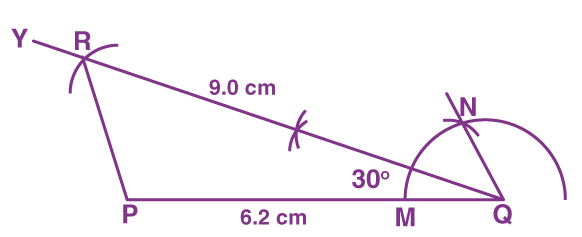Steps of Construction:

1. Draw a line segment PQ of length 6.2 cm

2. Taking Q as centre, draw an arc meeting PQ at point M

3. Taking M as centre and same radius, cut the arc at point N

4. Join QN

5. Now, bisect ∠NQP. Let QY be the bisector. QY makes an angle of 300 with PQ

6. Taking Q as centre and radius 9 cm cut an arc on QY and mark the point as R

7. Join PR

Therefore,

PQR is the required triangle

3. Construct a triangle using the given data:

(i) BC = 6.0 cm, ∠B = 600 and ∠C = 450

(ii) PQ = 6.2 cm, ∠P = 1050 and ∠Q = 450

(iii) DE = 5 cm, ∠D = 750 and ∠E = 600

Solution:

(i) BC = 6.0 cm, ∠B = 600 and ∠C = 450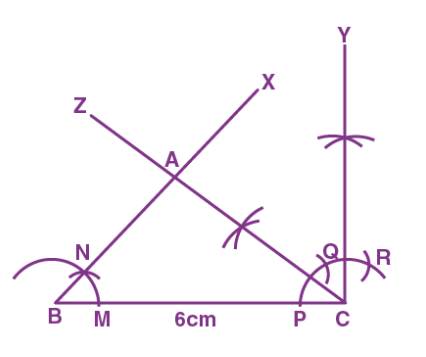Steps of Construction:

1. Draw a line segment BC of length 6 cm.

2. Taking B as centre, draw an arc meeting BC at point M

3. Taking M as centre and same radius, cut the arc at point N

4. Produce BN to BX.

5. BX makes an angle of 600 with BC

6. Taking C as centre, draw an arc meeting BC at point P

7. Taking P as centre and same radius, cut the arc at Q and taking Q as centre and same radius, cut the arc at R

8. Taking Q and R as centre, cut arcs and draw CY perpendicular to BC

9. Bisect ∠YCB. Let CZ be the bisector. Here, CZ makes an angle of 450 with BC

10. Mark the point as A, where BX and CZ meet each other

Hence, ABC is the required triangle

(ii) PQ = 6.2 cm, ∠P = 1050 and ∠Q = 450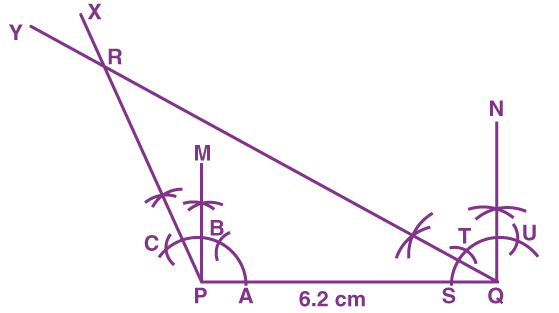Steps of Construction:

1. Draw a line segment PQ of length 6.2 cm

2. Taking P as centre, draw an arc meeting PQ at point A

3. Taking A as centre and same radius, cut the arc at point B and taking BQ as centre and same radius, cut the arc at point C

4. Now, taking B and C as centre, cut arcs and draw PM perpendicular to PQ

5. Let PX be the bisector to bisect ∠MPC. PX makes an angle of 1050 with PQ

6. Taking Q as centre, draw an arc meeting PQ at S

7. Taking S as centre and same radius, cut the arc at T and taking T as centre and same radius, cut the arc at U

8. Now, taking T and U as centre, cut the arcs and draw QN perpendicular to PQ

9. Let QY be the bisector to bisect ∠NQP. QY makes an angle of 450 with PQ

10. Mark the point as R, where PX and QY meet each other

Hence,

PQR is the required triangle

(iii) DE = 5 cm, ∠D = 750 and ∠E = 600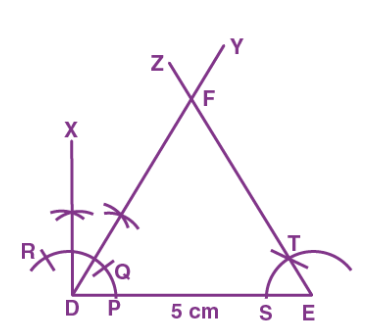Steps of Construction:

1. Draw a line segment DE of length 5 cm

2. Taking D as centre, draw an arc cutting DE at point P

3. Taking P as centre and same radius, cut the arc at Q and then taking Q as centre with same radius, cut the arc at point R

4. Taking Q and R as centre, bisect ∠RDQ thus formed to draw a ray XD

5. Let DY be the bisector, to bisect the ∠XDQ. DY makes an angle of 750 with DE

6. Taking E as centre, draw an arc meeting DE at point S

7. Taking S as centre and same radius cut the arc at point T

8. Produce ET to EZ.

9. EZ makes an angle of 600 with DE

10. Mark the point as F, where DY and EZ meet each other

Therefore,

DEF is the required triangle

4. Construct a right angled triangle in which:

(a) Side AB = 4.5 cm and hypotenuse AC = 7 cm

(b) Side DE = 6 cm and ∠E = 300, ∠D = 900

(c) QP = QR and hypotenuse PR = 7 cm

Solution:

(a) Side AB = 4.5 cm and hypotenuse AC = 7 cm

Steps of Construction:

1. Draw a line segment AB of length 4.5 cm

2. At B construct a ray BP such as ∠ABP = 900

3. Taking A as centre and radius 7 cm, draw an arc to cut BP at point C

4. Now, join AC

Hence,

ABC is the required triangle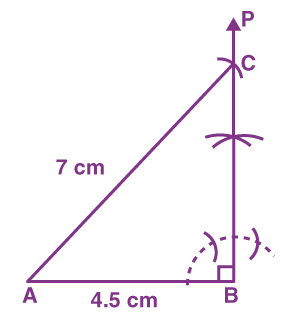(b) Side DE = 6 cm and ∠E = 300, ∠D = 900

Steps of Construction:

1. Draw a line segment DE of length 6 cm

2. At D, construct a ray DP such that ∠PDE = 900

3. Taking E as centre, draw ∠DEM of angle 300

4. Ray DP and ray EM intersect at point F

Therefore,

DEF is the required triangle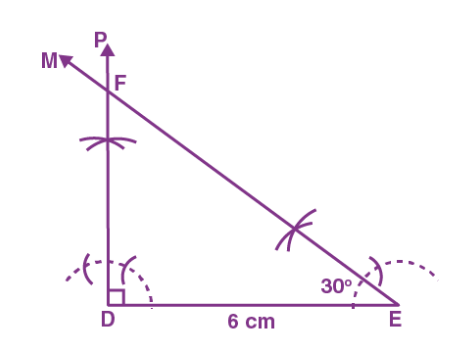(c) QP = QR and hypotenuse PR = 7 cm

Steps of Construction:

1. Draw a line segment PR of length 7 cm

2. Draw a ray PT such that ∠RPT = 450 and a ray RS such that ∠PRS = 450

3. Here, ray RS and ray PT meets at point Q

Therefore,

PQR is the required triangle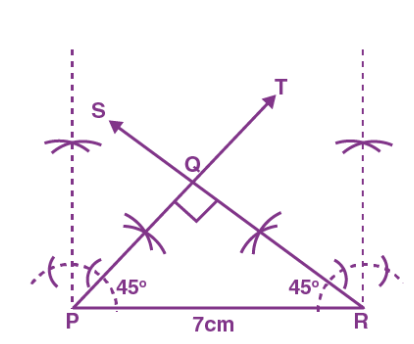Now,

In △PQR,

QP = QR …… (given)

∠QPR = ∠QRP …… (angles opposite to two equal sides are equal)

Here, hypotenuse PR = 7cm,

So,

∠PQR = 900

Hence,

∠QPR + ∠QRP = 900

∠QPR = ∠QRP = 450

5. Construct an isosceles triangle in which:

(a) AB = AC, BC = 6 cm and ∠B = 750

(b) XY = XZ, YZ = 5.5 cm and ∠X = 600

(c) PQ = QR, PR = 4.5 cm and ∠R = 600

Solution:

(a) AB = AC, BC = 6 cm and ∠B = 750

In △ABC,

AB = AC …… (given)

∠ACB = ∠ABC = 750

Steps of Construction:

1. Draw a line segment BC of length 6 cm

2. Construct ∠BCM = 750 and ∠CBN = 750

3. Ray CM and ray BN meets at a point A

Therefore,

ABC is a required triangle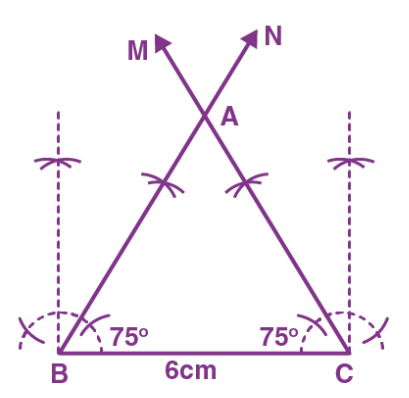(b) XY = XZ, YZ = 5.5 cm and ∠X = 600

In △XYZ,

XY = XZ ….. (given)

∠XZY = ∠XYZ ….. (1)

∠X = 600 …… (given)

Now,

∠X + ∠Y + ∠Z = 1800

600 + ∠Y + ∠Y = 1800 ……… [from (1)]

We get,

2∠Y = 1800 – 600

2∠Y = 1200

We get,

∠Y = 600 = ∠Z

Steps of Construction:

1. Draw a line segment YZ of length 5.5 cm

2. Construct an ∠YZP = 600 and ∠ZYQ = 600

3. Ray ZP and YQ meet at a point X

Therefore,

XYZ is the required triangle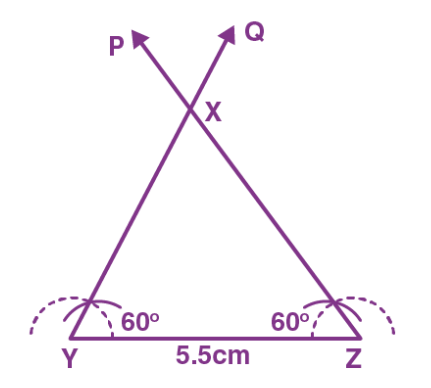(c) PQ = QR, PR = 4.5 cm and ∠R = 600

In △PQR,

PQ = QR ………. (given)

∠PRQ = ∠RPQ = 600

Steps of Construction:

1. Draw a line segment PR of length 4.5 cm

2. Construct ∠PRU = 600 and ∠RPV = 600

3. Ray RU and PV meet at a point Q

Therefore,

PQR is the required triangle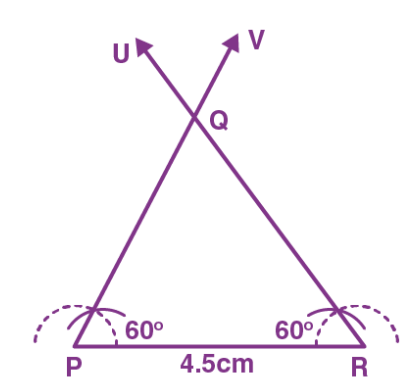6. Construct an isosceles triangle using the given data:

(i) Altitude RM = 5 cm and vertex ∠R = 1200

(ii) Altitude AD = 4 cm and vertex ∠A = 900

(iii) Altitude XT = 6.8 cm and vertex ∠X = 300

Solution:

(i) Altitude RM = 5 cm and vertex ∠R = 1200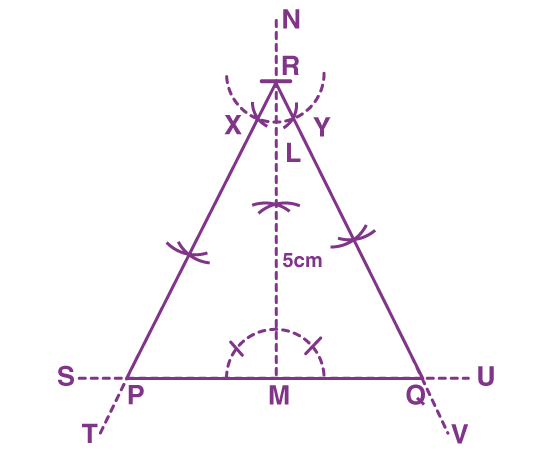Steps of Construction:

1. Draw a line segment SU of any length

2. Take a point M on SU

3. Through the point M on SU draw NM perpendicular to SU

4. Taking M as centre and radius 5 cm, draw an arc to cut NM at point R

5. Construct ∠MRP = ∠MRQ = (1 / 2) × 1200 = 600

(a)Taking R as centre, draw an arc cutting RM at point L

(b)Taking L as centre and same radius cut the arc at point X and Y

(c) Now, join RX and RY and produce them to T and V respectively. RT and RV make an angle of measure 600 with RM

(d) Mark the points as P and Q where RT and RV meet SU

Hence, PQR is the required triangle

(ii) Altitude AD = 4 cm and vertex ∠A = 900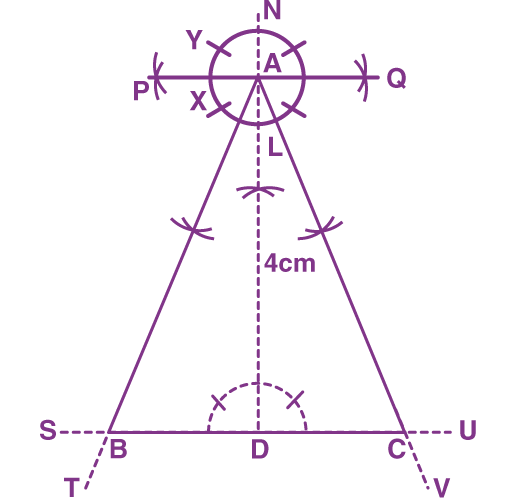Steps of Construction:

1. Draw a line segment SU of any length

2. Take a point D on SU

3. Through the point D on SU draw ND perpendicular to SU

4. Taking D as centre and radius 4 cm, draw an arc to cut ND at point A

5. Construct ∠DAB = ∠DAC = (1 / 2) × 900 = 450

(a) Taking A as centre, draw an arc cutting AD at point L

(b)Taking L as centre and same radius cut an arc at points X and Y

(c) Using X and Y, draw PQ perpendicular to AD

(d)Bisect ∠PAD and ∠QAD. Let AT and AV are the bisectors. AT and AV make an angle of 450 with AD

(e) Mark the points as B and C where AT and AV meet SU

Hence,

ABC is the required triangle

(iii) Altitude XT = 6.8 cm and vertex ∠X = 300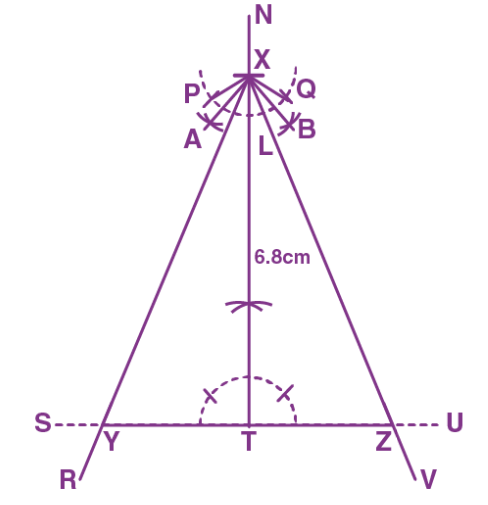Steps of Construction:

1. Draw a line segment SU of any length

2. Take a point T on SU

3. Through the point T on SU draw NT perpendicular to SU

4. Taking T as centre and radius 6.8 cm, draw an arc to cut NT at point X

5. Construct ∠TXY = ∠TXZ = (1 / 2) × 300 = 150

(a) Taking X as centre, draw an arc cutting XT at point L

(b) Taking L as centre and same radius, cut the arc at point P and Q

(c) Join PX and QX

(d) Bisect ∠PXT and ∠QXT. Let XA and XB be the bisectors

(e) Again bisect ∠AXT and ∠BXT. Let XR and XV be the bisectors. XR and XV make an angle of 150 with XT

(f) Mark the points as Y and Z where XR and XV meet SU

Hence, XYZ is the required triangle

7. Construct an isosceles right-angled triangle whose hypotenuse is of length 6 cm.

Solution:

Let △UVW be the isosceles right-angled triangle,

Right angled at point U

Hypotenuse VW = 6 cm

UV = UW

∠UWV = ∠UVW

∠U = 900

∠UWV + ∠UVW = 900

2 ∠UWV = 900

We get,

∠UWV = ∠UVW = 450

Steps of Construction:

1. Draw a line segment of length 6 cm

2. Construct ∠WVY = 450 and ∠VWX = 450

3. Ray VY and ray WX meet at point U

Hence,

UVW is the required triangle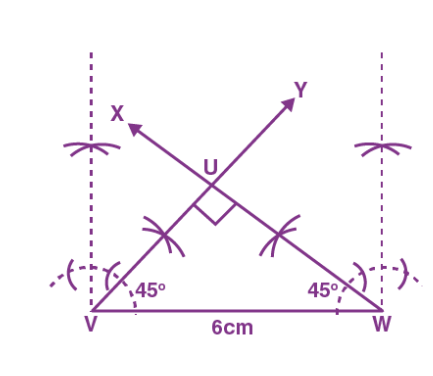8. Construct an equilateral triangle using the data:

(i) Altitude AD = 5 cm

(ii) Altitude PM = 3.6 cm

(iii) Altitude OM = 5.8 cm

Solution:

(i) Altitude AD = 5 cm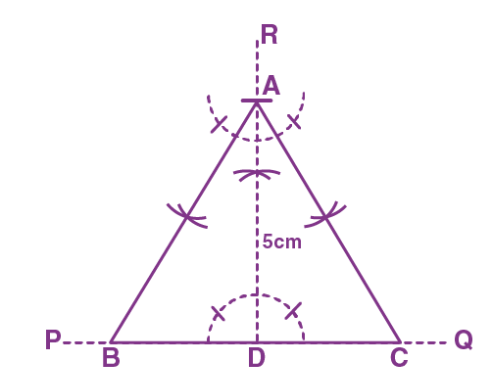Steps of Construction:

1. Draw a line segment PQ of any length

2. Through a point D on PQ, draw AD perpendicular to PQ such that AD = 5 cm

3. Through A, draw AB and AC making angles equal to 300 with AD and meeting PQ at points B and C respectively

Hence,

ABC is the required triangle

(ii) Altitude PM = 3.6 cm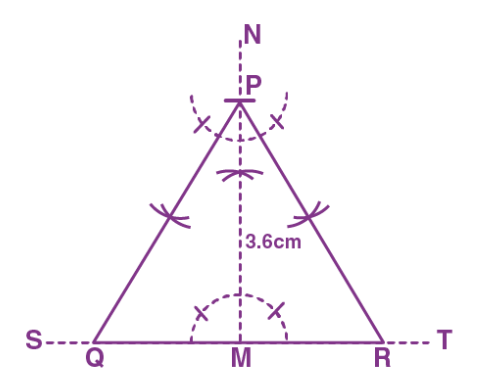Steps of Construction:

1. Draw a line segment ST of any length

2. Through a point M on ST, draw PM perpendicular to ST such that PM is of length 3.6 cm

3. Through P, draw PQ and PR making angles equal to 300 with PM and meeting ST at points Q and R respectively

Hence,

PQR is the required triangle

(iii) Altitude OM = 5.8 cmSteps of Construction:

1. Draw a line segment ST of any length

2. Through a point M on ST, draw PM perpendicular to ST such that OM is of length 5.8 cm

3. Through O, draw OQ and OR making angles equal to 300 with OM and meeting ST at Q and R respectively

Hence,

OQR is the required triangle

9. Construct a triangle using the following data:

(i) XY + YZ = 5.6 cm, XZ = 4.5 cm and ∠X = 450

(ii) PQ + PR = 10.6 cm, QR = 4.8 cm and ∠R = 450

(iii) DE + DF = 10.3 cm, EF = 6.4 cm and ∠E = 750

Solution:

(i) XY + YZ = 5.6 cm, XZ = 4.5 cm and ∠X = 450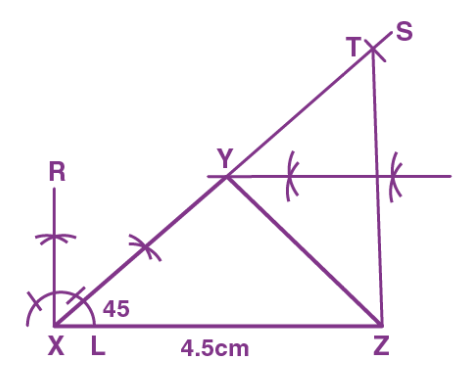Steps of Construction:

1. Draw a line segment XZ of length 4.5 cm

2. Taking X as centre, construct ∠SXZ = 450

3. Cut XT on XS such that XT = 5.6 cm

4. Join TZ

5. Draw a perpendicular bisector of TZ which cuts XT at point Y

6. Join YZ

Hence,

XYZ is the required triangle

(ii) PQ + PR = 10.6 cm, QR = 4.8 cm and ∠R = 450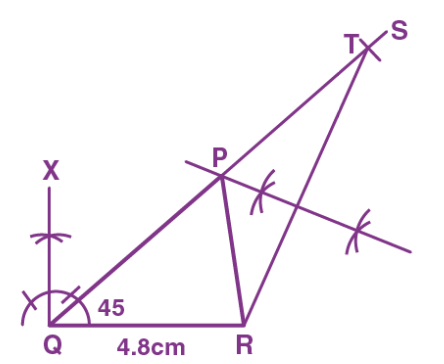Steps of Construction:

1. Draw a line segment QR of length 4.8 cm

2. Taking Q as centre, construct ∠SQR = 450

3. Cut QT on QS such that QT = 10.6 cm

4. Join TR

5. Draw perpendicular bisector of TR which cuts QT at point P

6. Now, join PR

Hence,

PQR is the required triangle

(iii) DE + DF = 10.3 cm, EF = 6.4 cm and ∠E = 750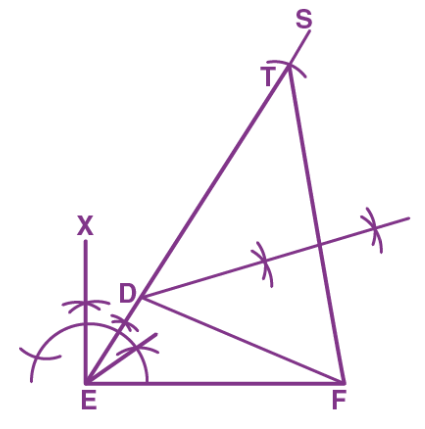Steps of Construction:

1. Draw a line segment EF of length 6.4 cm

2. Taking E as centre, construct ∠SEF = 750

3. Cut ET on ES such that ET = 10.3 cm

4. Join TF

5. Draw perpendicular bisector of TF which cut ET at point D

6. Join DF

Hence,

DEF is the required triangle

10. Construct a triangle using the given data:

(i) PQ – PR = 1.5 cm, QR = 6.0 cm and ∠Q = 750

(ii) AB – AC = 1.2 cm, BC = 6.0 cm and ∠B = 600

(iii) XY – XZ = 1.5 cm, YZ = 3.4 cm and ∠X = 450

Solution:

(i) PQ – PR = 1.5 cm, QR = 6.0 and ∠Q = 450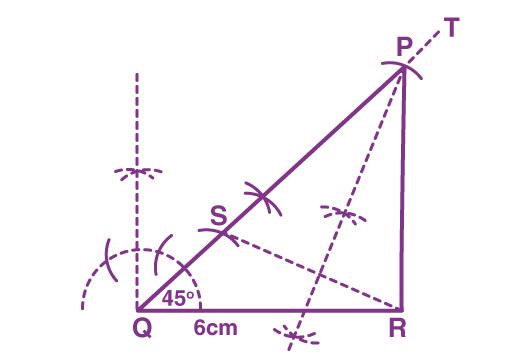Steps of Construction:

1. Draw a line segment QR of length 6 cm

2. Taking Q as centre, draw ∠TQR = 450

3. From Q, cut an arc of measure 1.5 cm on QT and name it as S

4. Now, join S and R

5. Draw a perpendicular bisector of SR which cuts QT at point P

6. Join PR

Therefore, PQR is the required triangle

(ii) AB – AC = 1.2 cm, BC = 6.0 cm and ∠B = 600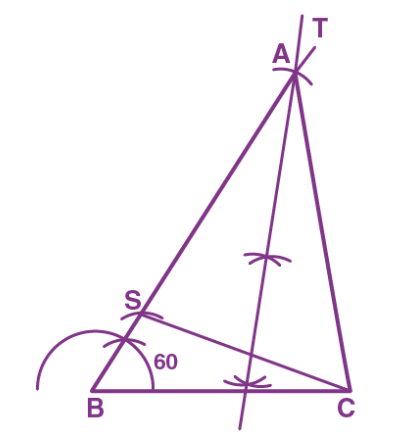Steps of Construction:

1. Draw a line segment BC of length = 6 cm

2. Taking B as centre, draw ∠TBC = 600

3. From B, cut an arc of measure 1.2 cm on BT and name it as point S

4. Now, join S and C

5. Draw a perpendicular bisector of SC which cuts BT at point A

6. Join AC

Therefore, ABC is the required triangle

(iii) XY – XZ = 1.5 cm, YZ = 3.4 cm and ∠X = 450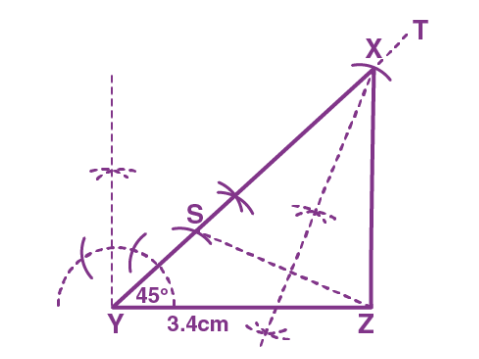Steps of Construction:

1. Draw a line segment YZ of length 3.4 cm

2. Taking Y as centre, draw ∠TYZ = 450

3. From Y, cut an arc of measure 1.5 cm on YT and name it as S

4. Now, join S and Z

5. Draw a perpendicular bisector of SZ which cuts YT at point X

6. Join XZ

Therefore, XYZ is the required triangle

11. Construct a triangle using the given data:

(i) Perimeter of triangle is 6.4 cm, and the base angles are 600 and 450

(ii) Perimeter of triangle is 9 cm, and the base angles are 600 and 450

(iii) Perimeter of triangle is 10.6 cm, and the base angles are 600 and 900

Solution:

(i) Perimeter of triangle is 6.4 cm, and the base angles are 600 and 450Steps of Construction:

1. Draw DE of measure 6.4 cm

2. Draw DP and EQ such that ∠PDE = 450 and ∠QED = 600

3. Draw AD and AE, the bisectors of angles PDE and QED respectively, intersecting each other at point A

4. Draw perpendicular bisectors of AD and AE, intersecting DE at B and C respectively

5. Now, join AB and AC

Therefore, ABC is the required triangle

(ii) Perimeter of triangle is 9 cm, and the base angles are 600 and 450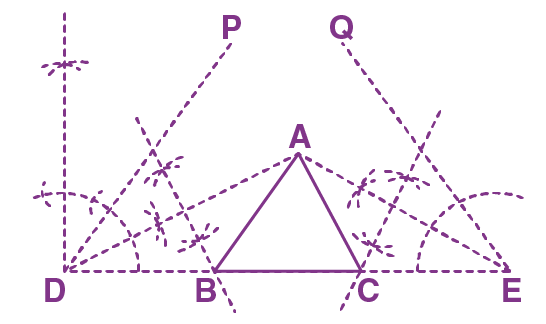Steps of Construction:

1. Draw DE of measure 9 cm

2. Draw DP and EQ, such that ∠PDE = 450 and ∠QED = 600

3. Draw AD and AE, the bisectors of angles PDE and QED respectively, intersecting each other at point A

4. Draw perpendicular bisectors of AD and AE, intersecting DE at B and C respectively

5. Now, join AB and AC

Therefore, ABC is the required triangle

(iii) Perimeter of triangle is 10.6 cm, and the base angles are 600 and 900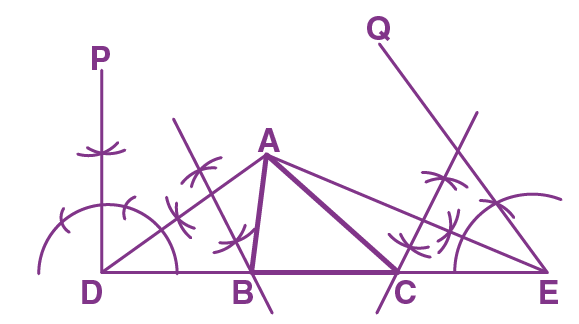Steps of Construction:

1. Draw DE of measure 10.6 cm

2. Draw DP and EQ such that ∠PDE = 900 and ∠QED = 600

3. Draw AD and AE, the bisectors of angles PDE and QED respectively, intersecting each other at point A

4. Draw perpendicular bisectors of AD and AE, intersecting DE at B and C respectively

5. Now, join AB and AC

Therefore, ABC is the required triangle

12. Construct a △XYZ with YZ = 7.5 cm, ∠Y = 600 and ∠Z = 450. Draw the bisectors of ∠Y and ∠Z. If these bisectors meet at O, measure angle YOZ.

Solution: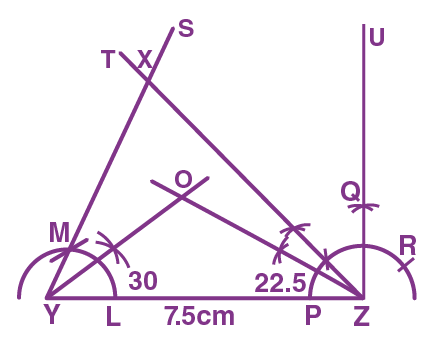Steps of Construction:

1. Draw a line segment YZ of length 7.5 cm

2. Taking Y as centre, draw an arc cutting YZ at point L

3. Taking L as centre and same radius cut the arc at M

4. Join Y and M. Produce YM to S. Now, YS makes an angle of 600 with YZ

5. With Z as centre, draw an arc cutting YZ at point P

6. Taking P as centre and same radius, cut the arc at Q, and then taking Q as centre and same radius cut the arc at R. Now, using Q and R, draw UZ perpendicular to YZ

7. Bisect ∠UZY. Let TZ be the bisector. TZ makes an angle of 450 with YZ

8. Bisect ∠SYZ and ∠TZY

9. Consider the point as O where the bisectors of ∠SYZ and ∠TZY meet

10. On measuring we get, ∠YOZ = 127.50

13. Construct a △RST with side ST = 5.4 cm, ∠RST = 600 and the perpendicular from R on ST = 3.0 cm

Solution: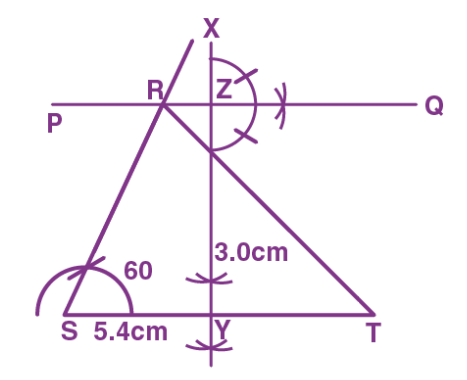Steps of Construction:

1. Draw a line segment ST of length 5.4 cm

2. Taking S as centre, draw XS such that ∠XST = 600

3. Draw a straight line PQ parallel to ST at a distance of 3 cm from ST

4. PQ meets XS at point R

5. Now, join RT

Therefore, ∠RST is the required triangle with angle 600

14. Construct a △PQR with ∠Q = 600, ∠R = 450 and the perpendicular from P to QR be 3.5 cm. Measure PQ.

Solution: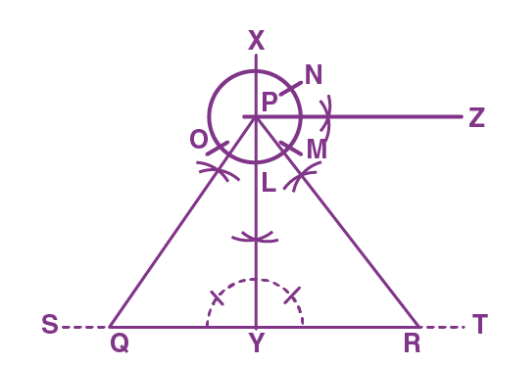Steps of Construction:

1. Draw a line segment ST of any length.

2. From any point Y on ST, draw XY perpendicular to ST.

3. Taking Y as centre and radius 3.5 cm, mark a point P on XY.

4. Taking P as centre, draw an arc cutting XY at point L.

5. With L as centre and same radius, cut the arc at O and M. With M as centre and same radius cut the arc at N

6. Draw PZ perpendicular to XY using M and N.

7. Bisect the angles OPY and ZPY making 300 and 450 angles with PY respectively.

(In triangle PQY, ∠PQY = 600, ∠QYP = 900, hence ∠QPY = 300 and in triangle PYR, ∠YRP = 450, ∠RYP = 900, hence ∠YPR = 450)

8. Now, join PQ and PR

9. PQR is the required triangle

10. On measuring, PQ = 4.1 cm.

15. Construct a △ABC, right angled at B with a perimeter of 10 cm and one acute angle of 600.

Solution: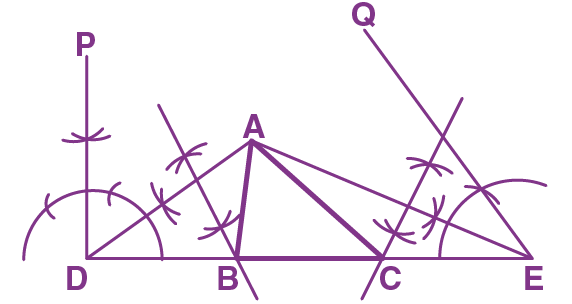Steps of Construction:

1. Draw DE of length 10 cm

2. Draw DP and EQ such that ∠PDE = 900 and ∠QED = 600.

3. Draw AD and AE, the bisectors of angles PDE and QED respectively, intersecting each other at point A.

4. Draw perpendicular bisectors AD and AE, intersecting DE at points B and C respectively.

5. Now, join AB and AC.

Hence, ABC is the required triangle.